# 20 Automatically Generating Stage Descriptions

Having annotated each line segment that makes up a rally stage route, can we use that information to help us create visual or even written, descriptions of a stage?

In terms of categorising turns in a way that’s meaningful in a rally context, let’s have a recap on what sorts of thing a rally navigator or co-driver is likely to be saying:

``embed_url("https://www.youtube.com/watch?v=KFjRj25uclQ")``

And let’s review what we actually mean by a corner…

``embed_url("https://www.youtube.com/watch?v=YXOYckDATWE")``

In terms of actual corners, the convexity interesting may be one of the more interesting properties. Finding a good way of representing variable radius turns (you did watch the “corners” video, didn’t you?!) will also be important if we want to be able to identify “tightens” or “lessens” (that is, “opens”) corner features. Any elevation changes in the vicinity of a corner are likely to be important if we are trying to characterise braking zone requirements…

## 20.1 Jemba Inertia Notes System

In a section entitled Using data to assess the suitability of a special stage, the FIA Rally Safety Guidelines 2020 suggest that as well as using historic information, the “Jemba System” for recording terrain data, including “the inclines, the dips, the bends and crests”. By also taking the road terrain (for example, gravel or asphalt) into account, and likely car classes, average speeds over the stage as well as highest speed and heaviest braking areas can also be determined. THe system can also report the margin for critical braking dist before stop (“how far you may carry on at competitive speed until you have to start braking to be able to stop at the stop control”) and the Jemba Safety Index (J/kg), the average kinetic energy of a car going through the corners.

The FIA Safety Guidelines illustrate how speeds might be visualised over a Google Earth map using a three colour scale (purple:over 150kph; red: over 90kph; yellow – 7590kph). The report also suggests that the Jemba system can predict the maximum cornering speed for any particular bend.

A Wikipedia page describing the Jemba Inertia Notes System gives examples of the descriptive and numerical labels that the Jemba system can generate as it converts odometry and accelerometer data into something rather more human understandable. A visual grading shows how descriptive labels correspond to turn angle.

## 20.2 Generating Stage Descriptions

Being able to display route profiles on a map as colour highlighted routes is very useful and could be made more so adding the data to a leaflet.js extension such as `Leaflet.Heightgraph`, which displays arbitrary traces (to integrate this in the current workflow properly would require the development of an extension to the R `leaflet` package).

Another possibility would be to try to automate the creation of text based route descriptions (“robot journalism”), which I’ve crudely explored in other contexts elsewhere. The It Gets Faster Now blog publishes stage descriptions for WRC stage routes, so this could be a good place to learn tropes from.

## 20.3 Load in Base Data

As ever, let’s load in our stage data and the elevation raster and create a demo map:

``````library(sf)
library(raster)

geojson_filename = 'montecarlo_2021.geojson'
``````## Reading layer `montecarlo_2021' from data source `/Users/tonyhirst/Documents/GitHub/visualising-rally-stages/montecarlo_2021.geojson' using driver `GeoJSON'
## Simple feature collection with 9 features and 2 fields
## geometry type:  LINESTRING
## dimension:      XY
## bbox:           xmin: 5.243488 ymin: 43.87633 xmax: 6.951953 ymax: 44.81973
## geographic CRS: WGS 84``````
``````stage_route_gj = geojsonio::geojson_json(geojson_sf[1,]\$geometry)

stage_tif = "stage_elevation.tif"

# Load in the previously saved image raster
elev_img = raster(stage_tif)

# Note we can pass in a file name or a raster object
elmat = raster_to_matrix(stage_tif)``````
``##  "Dimensions of matrix are: 382x565."``
``````demo_map = elmat %>%
progbar = FALSE)``````

Let’s also get a UTM projection of the stage routes:

``````# Detect the UTM zone as an EPSG code
lonlat2UTMzone = function(lonlat) {
utm = (floor((lonlat + 180) / 6) %% 60) + 1
if(lonlat > 0) {
utm + 32600
} else{
utm + 32700
}
}

get_utm_projection = function(routes){
# Keep track of the original proj4 string
old_crs = st_crs(geojson_sf[1,])\$proj4string

sample_location_x = st_coordinates(st_centroid(routes[1,]))
sample_location_y = st_coordinates(st_centroid(routes[1,]))

# Generate a new projection in the appropriate UTM zone
crs_zone = lonlat2UTMzone(c(sample_location_x,
sample_location_y))

new_proj4_string = st_crs(crs_zone)\$proj4string

# Transform the route to the UTM projection
utm_routes = st_transform(geojson_sf, crs=new_proj4_string)

utm_routes
# Or should we returned a named list
# e.g. including the original projection?
#list(utm_routes = utm_routes, orig_crs=old_crs)
}

utm_routes = get_utm_projection(geojson_sf)``````

## 20.4 Identifying Corners Based on `trajr` Step Gradients

Let’s start by trying to identify straight sections. Trivially, given that the stage route is given by a linestring made of straight line segments arranged at different angles relative to each other, every line segment is a straight. So what we really mean by a straight is a sequence of connected lines segments where each line segment is at an angle less than +/- 5 degrees to the to segment before it, for example, where the start of the straight has a preceding segment more than +/- 5 degrees to it (for example) and the end of the straight has a following line segment at more than +/- five degrees to it.

Let’s use the `trajr` stepangle to try to categorise straights:

``````library(trajr)

trj <- TrajFromCoords(as.data.frame(st_coordinates(utm_routes[1,])))

# Remove duplicate x,y rows - assume non-crossing route]
# Should probably flag this removal via a warning
trj =  trj[!duplicated(trj[,c('x','y')]),]

# displacement is a complex number, so we can get the actual distance:
trj\$distance = Mod(trj\$displacement)

# Find the accumulated distance at each step
trj\$cum_dist = cumsum(trj\$distance)

# Step angle in radians relative to previous
trj\$stepangle = c(0, TrajAngles(trj, compass.direction = NULL) * 180 / pi, NA)

trj\$cumstepangle = cumsum(c(0, TrajAngles(trj, compass.direction = NULL) * 180 / pi, NA))

trj\$stepheading = c(TrajAngles(trj, compass.direction = 0)* 180 / pi, NA)

# Find the gradient of the accumulated angle

``````g = ggplot(data=trj,
aes(x=x, y=y)) + geom_path(color='grey') + coord_sf()

g + geom_point( size=0.2, color='blue',
geom_point(size=0.2,
color='red')``````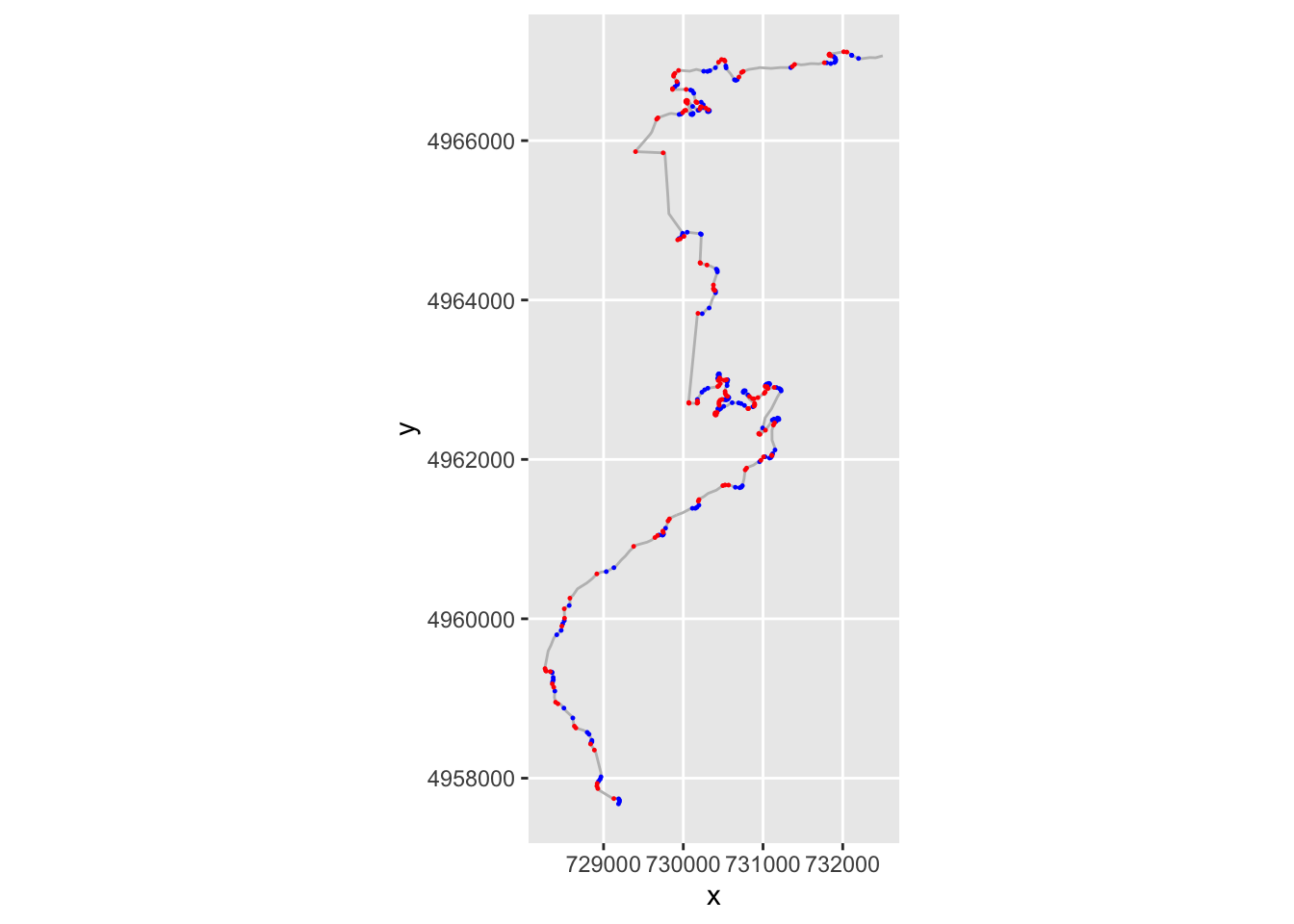If we have several consecutive points with a gradient of the same sign, then we might consider them to be part of the same corner, albeit one that maybe tightens or opens. If the gradients of consecutive segments have a different sign, then we have a change of direction from e.g. left to right or right to left.

We can create a flag that identifies when the sign changes across consecutive rows of the step_gradient column. We can check this by lagging the contents of the step_gradient column by one step using the `dplyr::lag()` function and comparing its sign to the sign of the unlagged value. If we then dplyr::lead() the result, we can see if a change in direction happens at the end of a step:

``````trj = trj %>%

``````##   step_gradient dirChange
## 1    0.02722146     FALSE
## 2    0.43059007     FALSE
## 3    1.18714584     FALSE
## 4    1.94367547     FALSE
## 5    1.42337267      TRUE
## 6   -0.07676768     FALSE``````

Let’s plot points on the route where we seem to have a direction change:

``````g +
geom_point( size=0.2, color='blue',
data=trj[trj\$dirChange,]) +
theme(axis.text.x = element_text(angle = 45))``````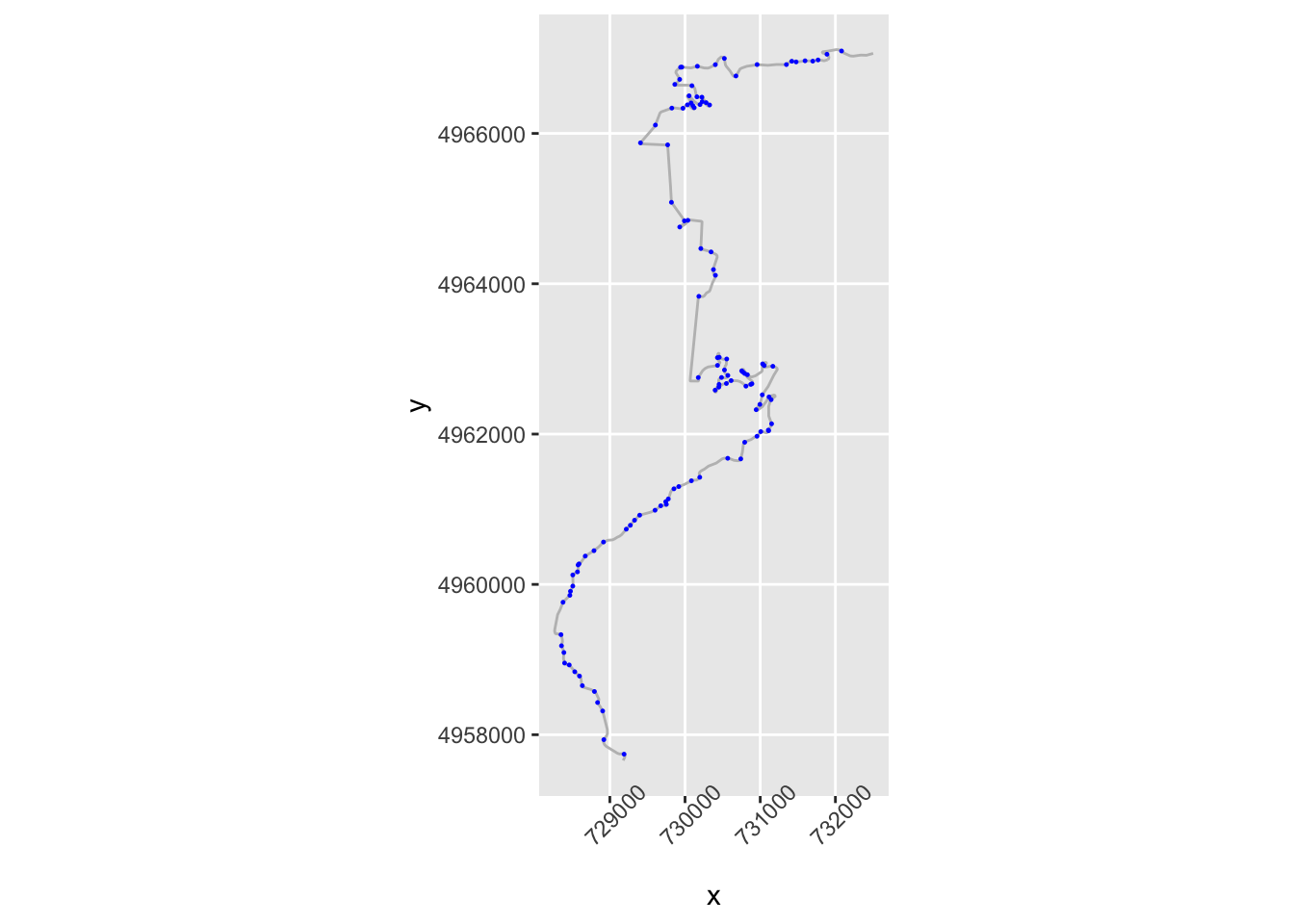Let’s zoom in a bit:

``````# Zoom in on part of the route
zoom1 = trj\$y>4964000 & trj\$y<4965000

g1 = ggplot(data=trj[zoom1, ],
aes(x=x, y=y)) + geom_path(color='grey') + coord_sf()

g1 +  geom_point( size=0.2, color='blue',
data=trj[zoom1 & trj\$dirChange,]) +
theme(axis.text.x = element_text(angle = 45))``````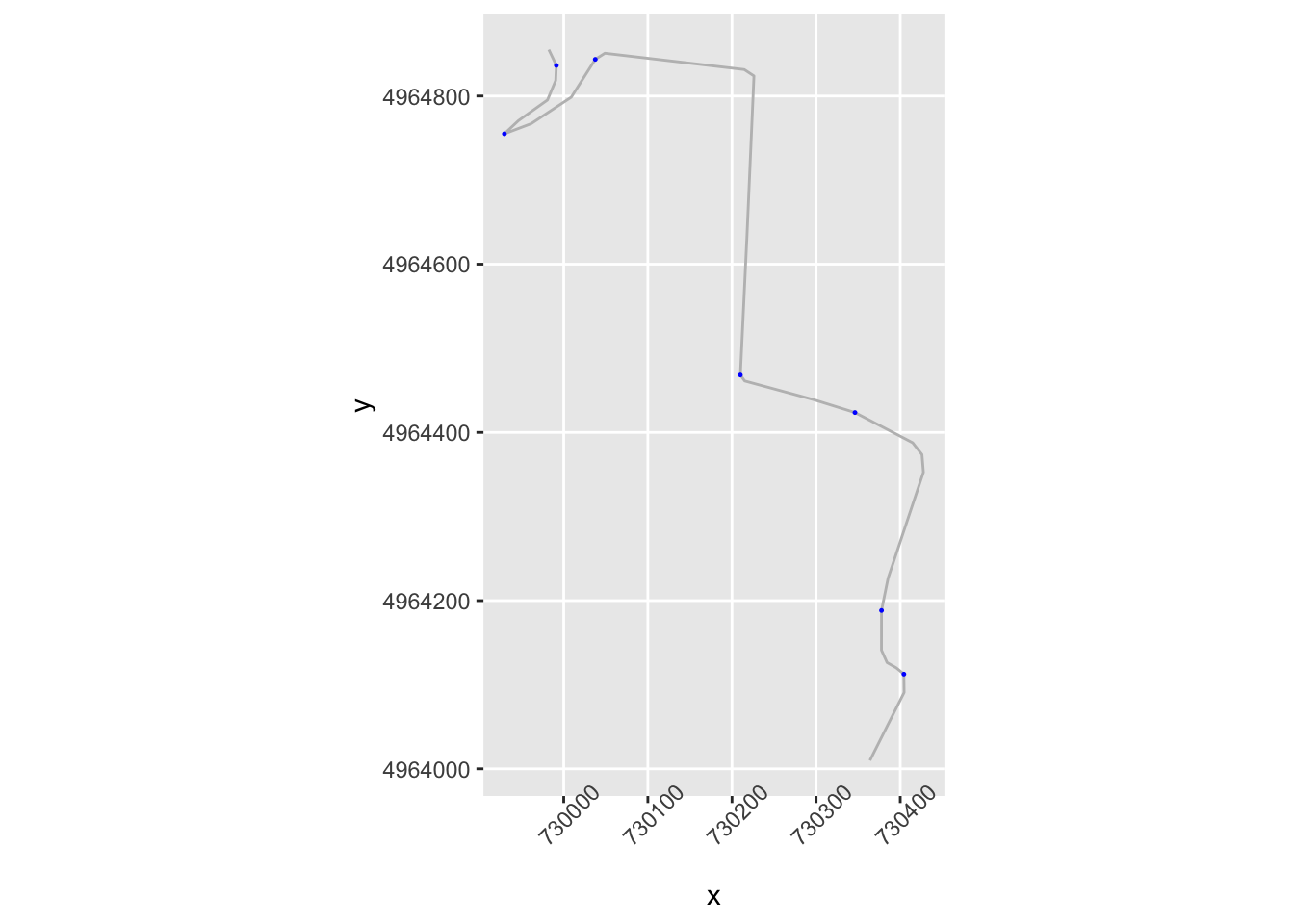We notice that we seem to be missing some corners that are perhaps not direction changes, but significant angle changes in the same direction. And we also notice a direction change flagged in what appears to be the middle of a straight.

Let’s look at the apparent turn in the middle of the straight first. If we have opposing angles from one segment to the next, then we currently class the first point as a corner because the direction has changed. But we aren’t really interested in slight direction changes or even slight direction changes in the same direction.

``````slight_gradient = 0.35
large_angle = 20

trj = trj %>%
(stepangle < large_angle))

ggplot(data=trj[zoom1, ],
aes(x=x, y=y)) + geom_path(color='grey') + coord_sf() +
geom_point( size=1, aes(color = straightish)) +
theme(axis.text.x = element_text(angle = 45))``````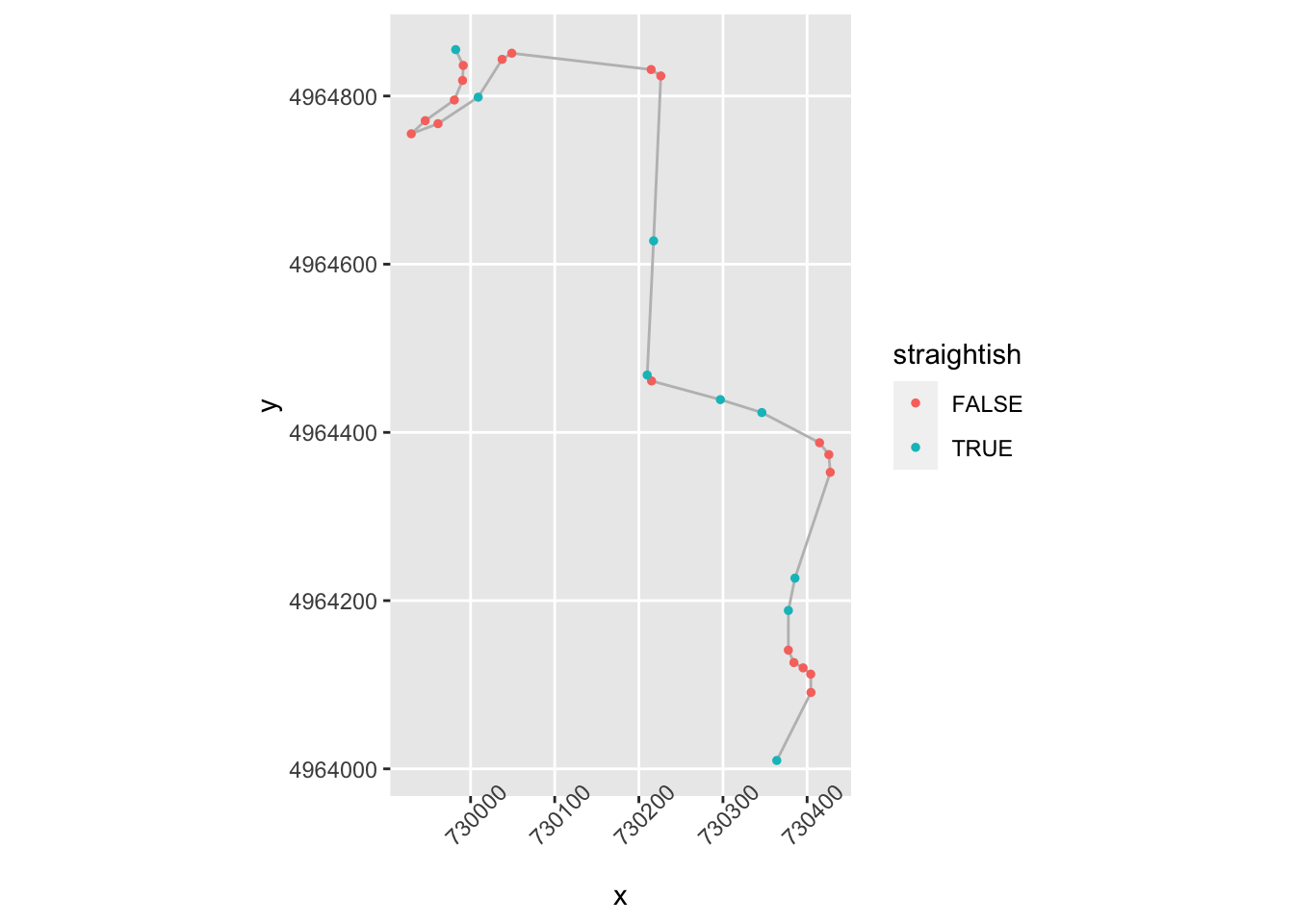We now want to identify the first node in a close sequence of nodes that identify a turn that doesn’t change direction.

Which is to say we want a not straightish node whose next node is nearby and not straightish and in the same direction, or not nearby:

``````# Close distance threshold
closeby = 25

trj = trj %>%
mutate(nearby = (distance < closeby) ) %>%
mutate(firstish = !straightish &
((nearby & !lag(straightish) & lag(dirChange)) |
(!nearby) ) )

ggplot(data=trj[zoom1, ],
aes(x=x, y=y)) + geom_path(color='grey') + coord_sf() +
geom_point( size=1, aes(color = firstish)) +
theme(axis.text.x = element_text(angle = 45))``````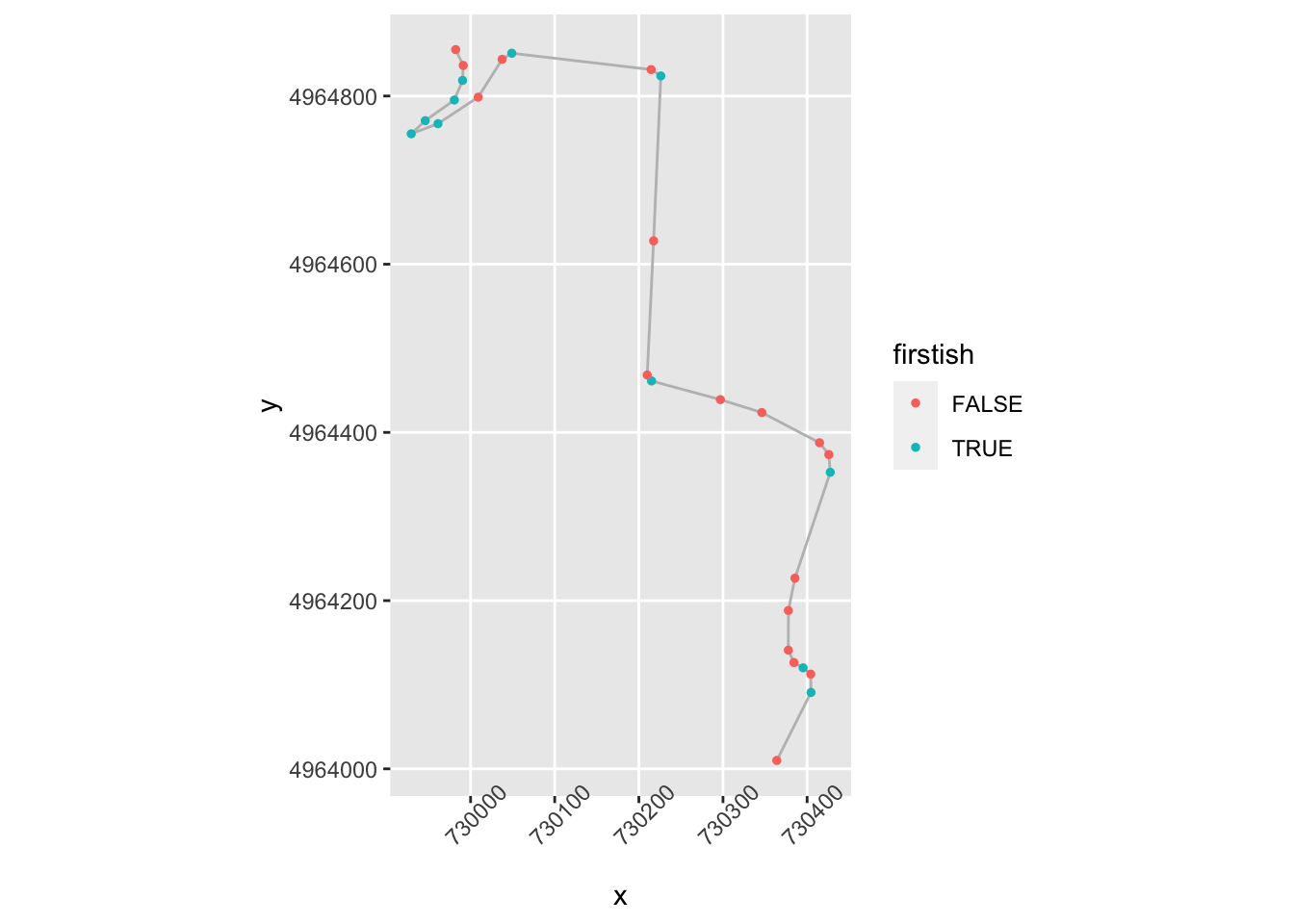That’s identifying some of the corners, but what’s going on top left?

``````zoom2 = zoom1 & trj\$x<730100

ggplot(data=trj[zoom2,],
aes(x=x, y=y)) + geom_path(color='grey') + coord_sf() +
geom_point( size=1, aes(color = firstish)) +
theme(axis.text.x = element_text(angle = 45))``````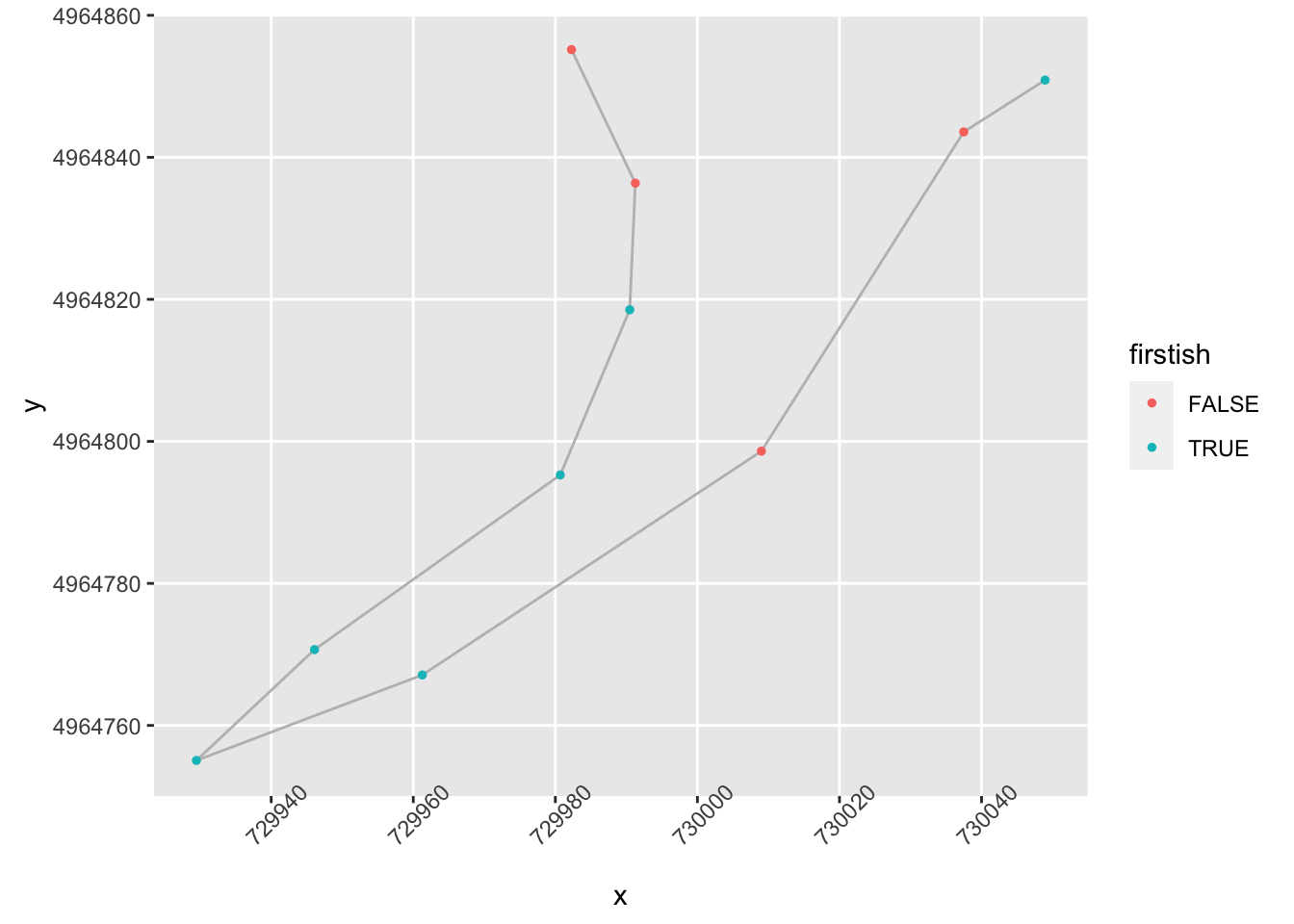Ah, ok, maybe we could categorise those as turns, but perhaps gentle ones. We maybe need to flag these as “flowing” if they aren’t too tight and have a long run in and out:

``````tight_gradient = 0.5

trj = trj %>%
mutate(flowing = !nearby & !lead(nearby) &
!straightish & !significant )

ggplot(data=trj[zoom2,],
aes(x=x, y=y)) + geom_path(color='grey') + coord_sf() +
geom_point( size=3, aes(color = flowing,
shape = dirChange)) +
theme(axis.text.x = element_text(angle = 45))``````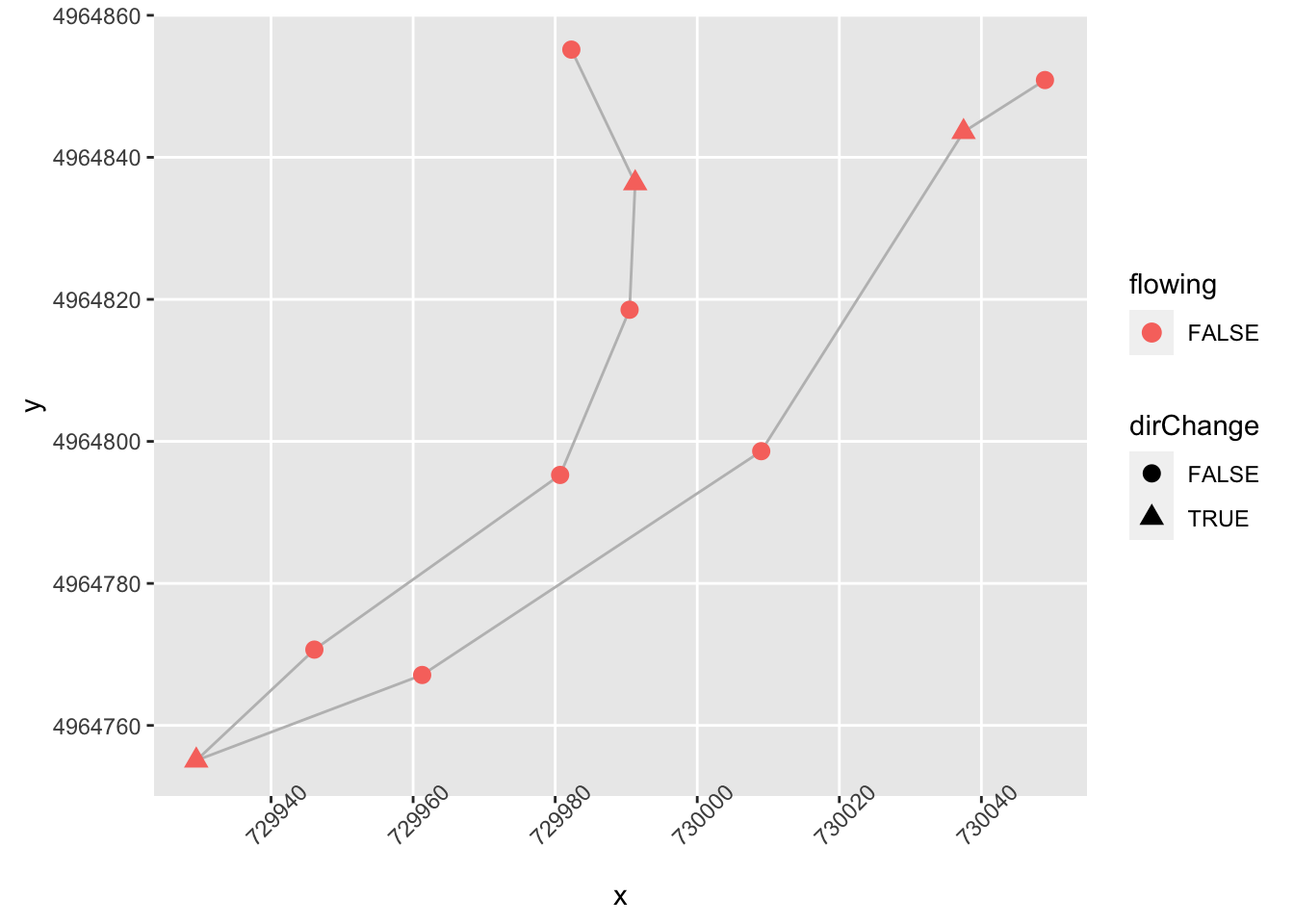We can also tweak firstish to recognise significance:

``````trj = trj %>%
mutate(firstish = (significant & !lag(significant)) |
(!straightish &
((nearby & !lag(straightish) & lag(dirChange)) |
(!nearby) ) )) %>%
# Desensitise firstish
mutate(firstish = firstish & !(lag(firstish)))``````

And how about over a wider part of the stage?

``````ggplot(data=trj[zoom1, ],
aes(x=x, y=y)) + geom_path(color='grey') + coord_sf() +
geom_point( size=2, aes(color = firstish,
shape = flowing)) +
theme(axis.text.x = element_text(angle = 45))``````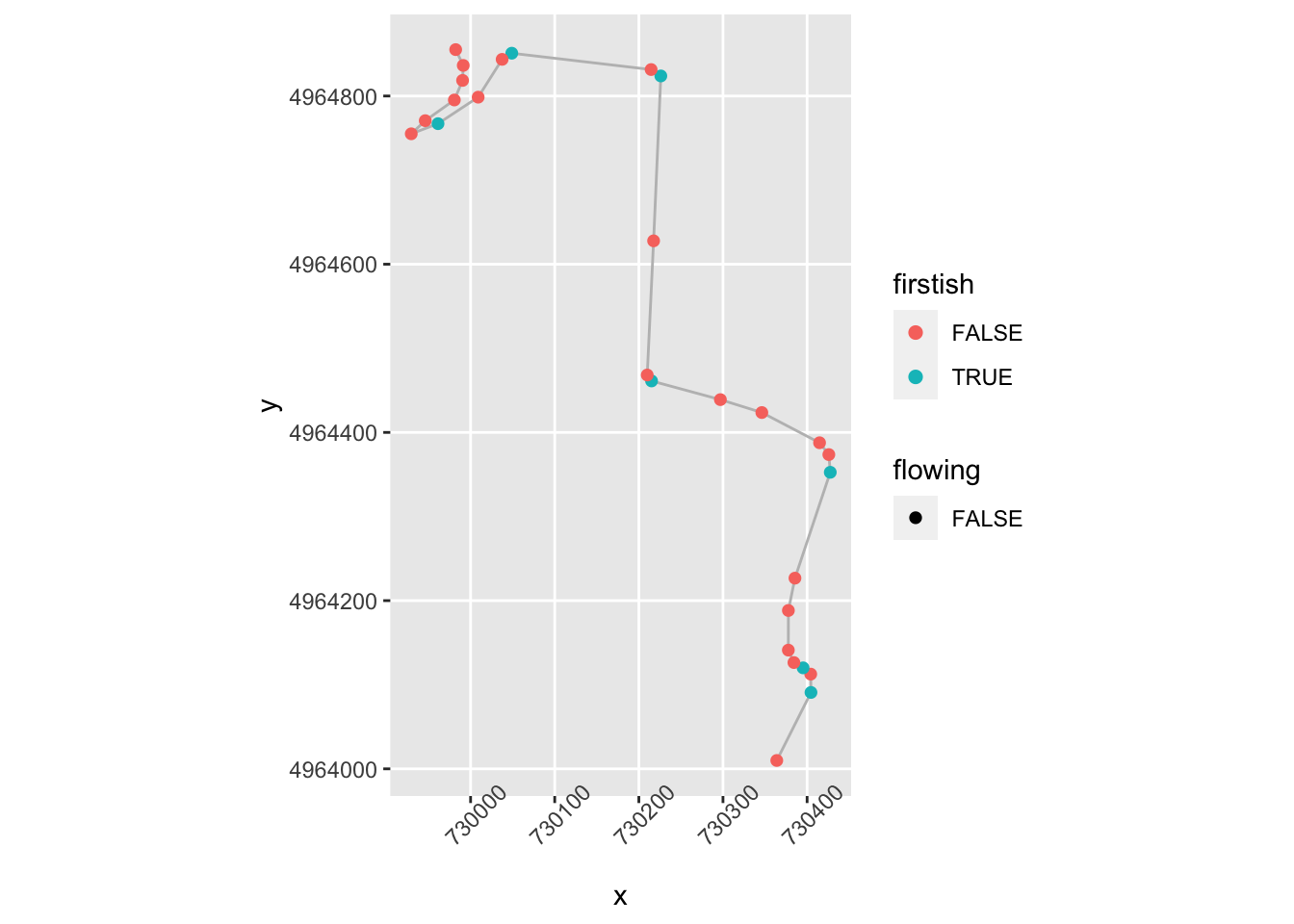We could also then create a !flowing & firstish flag to provide better indexing of the starts of corners.

We’d need to test the approach on further stages to see whether it gives false positives or false negatives on identifying various corners, and we’d maybe also want to try to identify tightens and opens.

Let’s have a go at tightens. At a first approximation, a corner tightens if a node isn’t firstish, is above a certain gradient, is close to the previous step and the magnitude of the gradient has increased and is the same sign as the previous step:

``````trj = trj %>%
mutate(tightens = !firstish & significant & lead(nearby) &

ggplot(data=trj[zoom1, ],
aes(x=x, y=y)) + geom_path(color='grey') + coord_sf() +
geom_point( size=2, aes(color = tightens,
shape = firstish)) +
theme(axis.text.x = element_text(angle = 45))``````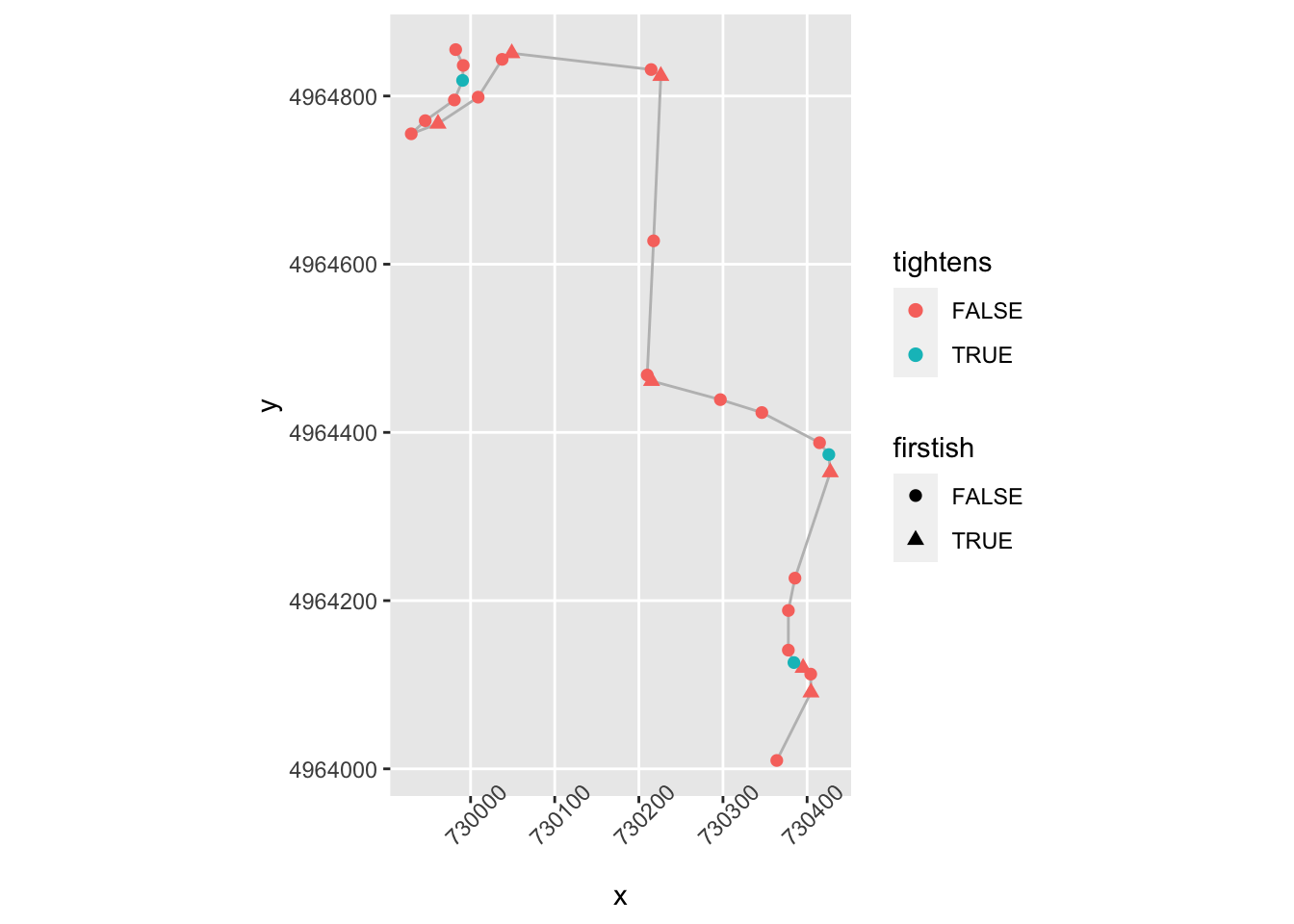The corner opens (“lessens”) if a node isn’t firstish, is above a certain gradient, is close to the previous step and the gradient is in the same direction and has decreased:

``````trj = trj %>%
mutate(opens = !firstish & significant & lead(nearby) &

ggplot(data=trj[zoom1, ],
aes(x=x, y=y)) + geom_path(color='grey') + coord_sf() +
geom_point( size=2, aes(color = opens,
shape = firstish)) +
theme(axis.text.x = element_text(angle = 45))``````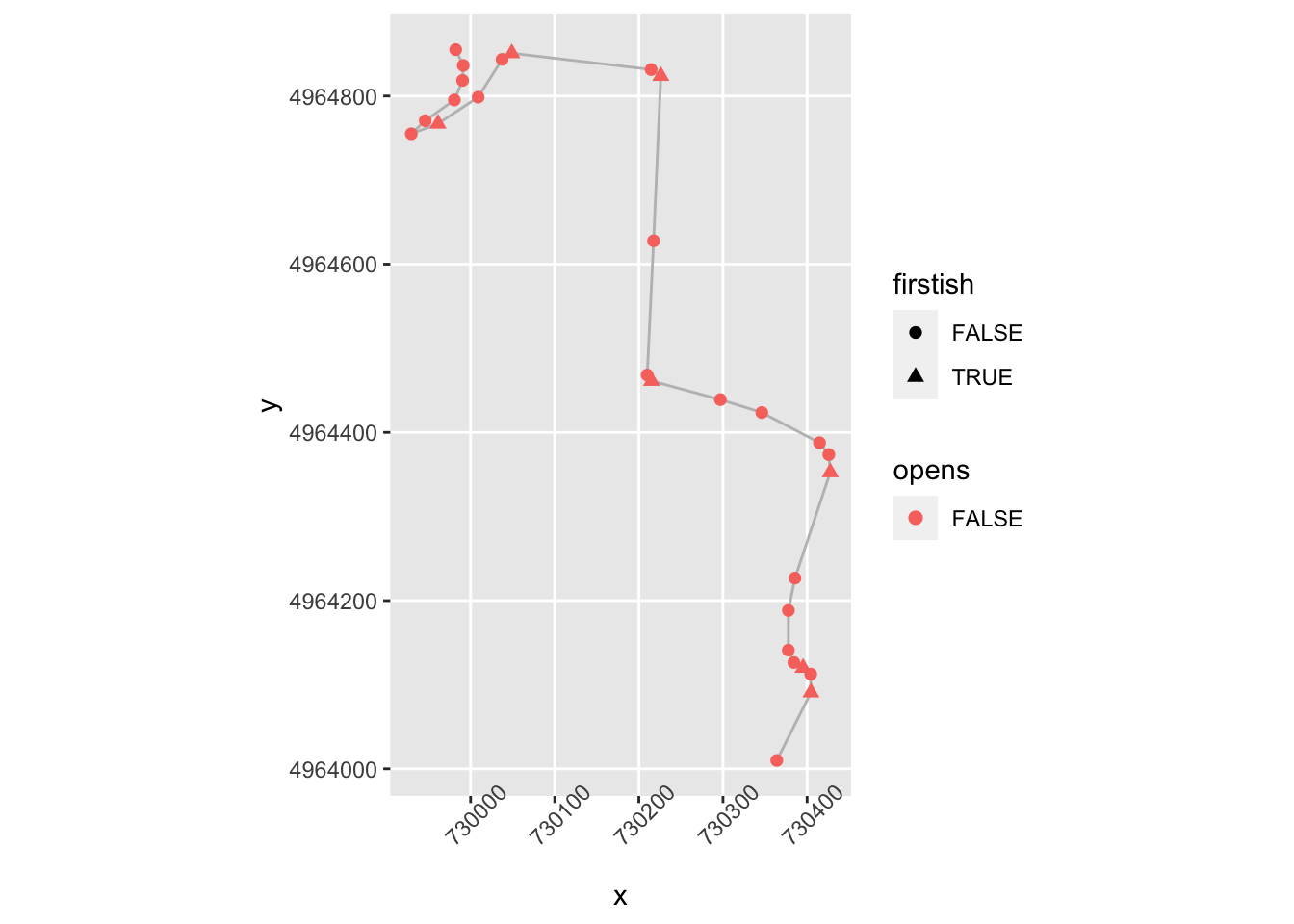We might also try for “continues” if the angle is about the same gradient but not tightening.

## 20.5 Identifying Straights

One of the important considerations when assessing a stage is identifying the fast areas of the stage represented by significant straights. So how can we identify a straight?

One way might be to define a simple rule that identifies a straight as a sequence of `straightish` segments that appear between segments that are not `straightish`.

We can create such groupings by flagging the presence of the start of a potential run of straightish segments. We can then number each start and propagate this run number down the following segments until the next straight segment is reached. We can then number all non-straighish elements with a “not straight” count number (e.g. -1).

``````trj = trj %>% mutate(startStraight = lag(!straightish, default=TRUE) & straightish) %>%
mutate(strcount = cumsum(startStraight))

trj\$strcount[!trj\$straightish] = -1

trj[, c('straightish', 'startStraight', 'strcount', 'distance')]``````
``````##     straightish startStraight strcount   distance
## 1          TRUE          TRUE        1   0.000000
## 2         FALSE         FALSE       -1  22.576096
## 3         FALSE         FALSE       -1  27.939693
## 4         FALSE         FALSE       -1  21.006147
## 5         FALSE         FALSE       -1  20.385688
## 6          TRUE          TRUE        2  21.776253
## 7         FALSE         FALSE       -1  39.225069
## 8          TRUE          TRUE        3  28.173437
## 9          TRUE         FALSE        3 176.799671
## 10        FALSE         FALSE       -1  34.213905
## 11        FALSE         FALSE       -1  33.435610
## 12        FALSE         FALSE       -1  33.180079
## 13         TRUE          TRUE        4  32.988458
## 14        FALSE         FALSE       -1  32.653388
## 15        FALSE         FALSE       -1  30.955250
## 16         TRUE          TRUE        5  52.133335
## 17         TRUE         FALSE        5 254.411703
## 18        FALSE         FALSE       -1  41.724705
## 19        FALSE         FALSE       -1  89.520784
## 20        FALSE         FALSE       -1  15.962401
## 21        FALSE         FALSE       -1  16.560182
## 22        FALSE         FALSE       -1  18.258302
## 23         TRUE          TRUE        6  85.379552
## 24         TRUE         FALSE        6  32.448662
## 25         TRUE         FALSE        6  51.783361
## 26        FALSE         FALSE       -1 102.162288
## 27        FALSE         FALSE       -1  29.056251
## 28         TRUE          TRUE        7  30.779766
## 29        FALSE         FALSE       -1  74.149638
## 30         TRUE          TRUE        8  30.943330
## 31         TRUE         FALSE        8  85.171697
## 32        FALSE         FALSE       -1  51.410943
## 33         TRUE          TRUE        9  65.299139
## 34        FALSE         FALSE       -1  33.110534
## 35        FALSE         FALSE       -1  34.904722
## 36         TRUE          TRUE       10  62.641285
## 37         TRUE         FALSE       10  79.381427
## 38         TRUE         FALSE       10  50.463921
## 39        FALSE         FALSE       -1  45.234343
## 40         TRUE          TRUE       11  24.320789
## 41        FALSE         FALSE       -1  28.123378
## 42         TRUE          TRUE       12  29.680685
## 43        FALSE         FALSE       -1  62.350861
## 44        FALSE         FALSE       -1   8.561852
## 45        FALSE         FALSE       -1  19.176687
## 46        FALSE         FALSE       -1  51.746203
## 47        FALSE         FALSE       -1   9.929782
## 48        FALSE         FALSE       -1  25.986880
## 49         TRUE          TRUE       13  24.790260
## 50         TRUE         FALSE       13 200.394033
## 51         TRUE         FALSE       13  79.003846
## 52         TRUE         FALSE       13 101.740035
## 53         TRUE         FALSE       13  51.526089
## 54        FALSE         FALSE       -1  78.114767
## 55         TRUE          TRUE       14  25.987677
## 56        FALSE         FALSE       -1  28.443655
## 57         TRUE          TRUE       15  30.354745
## 58        FALSE         FALSE       -1  45.272690
## 59         TRUE          TRUE       16  30.072585
## 60        FALSE         FALSE       -1 118.383431
## 61         TRUE          TRUE       17  18.073097
## 62        FALSE         FALSE       -1  57.463884
## 63         TRUE          TRUE       18  22.527126
## 64        FALSE         FALSE       -1  71.698716
## 65         TRUE          TRUE       19  19.073636
## 66         TRUE         FALSE       19  33.669783
## 67         TRUE         FALSE       19 100.195791
## 68         TRUE         FALSE       19  54.629746
## 69         TRUE         FALSE       19  81.943726
## 70         TRUE         FALSE       19  82.769644
## 71         TRUE         FALSE       19  88.612474
## 72         TRUE         FALSE       19  64.939488
## 73         TRUE         FALSE       19  58.082143
## 74         TRUE         FALSE       19  31.622830
## 75         TRUE         FALSE       19  44.266782
## 76        FALSE         FALSE       -1  32.481888
## 77         TRUE          TRUE       20  35.795692
## 78         TRUE         FALSE       20  93.700955
## 79         TRUE         FALSE       20  74.648418
## 80         TRUE         FALSE       20  87.518624
## 81         TRUE         FALSE       20  39.425264
## 82        FALSE         FALSE       -1  34.453996
## 83         TRUE          TRUE       21  22.856731
## 84         TRUE         FALSE       21 155.837831
## 85         TRUE         FALSE       21  59.493071
## 86         TRUE         FALSE       21  54.692563
## 87        FALSE         FALSE       -1  41.676102
## 88         TRUE          TRUE       22  20.134693
## 89        FALSE         FALSE       -1  42.504888
## 90        FALSE         FALSE       -1  10.319788
## 91        FALSE         FALSE       -1  10.082093
## 92        FALSE         FALSE       -1  19.947176
## 93        FALSE         FALSE       -1  16.696573
## 94         TRUE          TRUE       23  15.147739
## 95        FALSE         FALSE       -1  36.072187
## 96         TRUE          TRUE       24  30.108972
## 97        FALSE         FALSE       -1  66.401404
## 98        FALSE         FALSE       -1  31.078879
## 99         TRUE          TRUE       25  32.097088
## 100        TRUE         FALSE       25  70.861823
## 101        TRUE         FALSE       25  78.076153
## 102        TRUE         FALSE       25 107.148370
## 103        TRUE         FALSE       25  30.689626
## 104       FALSE         FALSE       -1  37.068369
## 105       FALSE         FALSE       -1  23.433769
## 106       FALSE         FALSE       -1  35.736928
## 107        TRUE          TRUE       26  10.809889
## 108       FALSE         FALSE       -1  41.278750
## 109       FALSE         FALSE       -1  21.243105
## 110        TRUE          TRUE       27  25.242190
## 111        TRUE         FALSE       27  48.187023
## 112        TRUE         FALSE       27  66.565053
## 113        TRUE         FALSE       27 110.173299
## 114        TRUE         FALSE       27  99.330798
## 115       FALSE         FALSE       -1  29.736978
## 116        TRUE          TRUE       28  43.479484
## 117        TRUE         FALSE       28  37.302236
## 118       FALSE         FALSE       -1  52.131224
## 119       FALSE         FALSE       -1  54.558722
## 120       FALSE         FALSE       -1  22.235672
## 121        TRUE          TRUE       29  22.448675
## 122        TRUE         FALSE       29  89.531050
## 123       FALSE         FALSE       -1 112.844215
## 124       FALSE         FALSE       -1  28.208403
## 125        TRUE          TRUE       30  29.689502
## 126        TRUE         FALSE       30  64.716386
## 127        TRUE         FALSE       30  47.552609
## 128       FALSE         FALSE       -1  40.762039
## 129       FALSE         FALSE       -1  24.293957
## 130       FALSE         FALSE       -1  55.480416
## 131        TRUE          TRUE       31  18.609341
## 132       FALSE         FALSE       -1  57.742931
## 133       FALSE         FALSE       -1  17.900876
## 134       FALSE         FALSE       -1  24.657085
## 135       FALSE         FALSE       -1   3.910547
## 136       FALSE         FALSE       -1   6.281999
## 137       FALSE         FALSE       -1  22.208888
## 138       FALSE         FALSE       -1  56.046544
## 139        TRUE          TRUE       32  18.390054
## 140        TRUE         FALSE       32 111.373808
## 141        TRUE         FALSE       32 125.767864
## 142        TRUE         FALSE       32  66.618897
## 143       FALSE         FALSE       -1  29.975148
## 144       FALSE         FALSE       -1  28.384868
## 145       FALSE         FALSE       -1  40.239085
## 146       FALSE         FALSE       -1  13.838288
## 147       FALSE         FALSE       -1  15.598591
## 148       FALSE         FALSE       -1  46.091581
## 149       FALSE         FALSE       -1  23.500236
## 150        TRUE          TRUE       33  24.854582
## 151        TRUE         FALSE       33  42.943277
## 152        TRUE         FALSE       33  59.516315
## 153       FALSE         FALSE       -1  27.085080
## 154       FALSE         FALSE       -1  86.155677
## 155       FALSE         FALSE       -1  13.499961
## 156       FALSE         FALSE       -1   6.781840
## 157        TRUE          TRUE       34  17.789179
## 158        TRUE         FALSE       34  68.720036
## 159        TRUE         FALSE       34  46.517087
## 160        TRUE         FALSE       34  84.947862
## 161        TRUE         FALSE       34 134.380640
## 162        TRUE         FALSE       34 170.177457
## 163       FALSE         FALSE       -1  88.585179
## 164       FALSE         FALSE       -1  17.936243
## 165       FALSE         FALSE       -1  21.604093
## 166       FALSE         FALSE       -1  39.653677
## 167       FALSE         FALSE       -1  27.667933
## 168       FALSE         FALSE       -1  79.679163
## 169       FALSE         FALSE       -1  13.988367
## 170       FALSE         FALSE       -1  13.865176
## 171       FALSE         FALSE       -1  22.728199
## 172       FALSE         FALSE       -1  20.813460
## 173       FALSE         FALSE       -1   8.714071
## 174       FALSE         FALSE       -1  10.860020
## 175       FALSE         FALSE       -1  25.095519
## 176       FALSE         FALSE       -1  18.796776
## 177        TRUE          TRUE       35  18.592286
## 178       FALSE         FALSE       -1  73.171879
## 179        TRUE          TRUE       36  18.603466
## 180        TRUE         FALSE       36  95.265566
## 181       FALSE         FALSE       -1  50.723320
## 182       FALSE         FALSE       -1  20.362347
## 183        TRUE          TRUE       37  46.707566
## 184       FALSE         FALSE       -1  26.893695
## 185       FALSE         FALSE       -1  62.138755
## 186       FALSE         FALSE       -1  15.898919
## 187       FALSE         FALSE       -1  15.380670
## 188       FALSE         FALSE       -1   8.399971
## 189        TRUE          TRUE       38  19.153761
## 190        TRUE         FALSE       38  32.612671
## 191        TRUE         FALSE       38  69.641579
## 192       FALSE         FALSE       -1  77.022940
## 193       FALSE         FALSE       -1  17.922554
## 194       FALSE         FALSE       -1  17.353440
## 195        TRUE          TRUE       39  17.998275
## 196        TRUE         FALSE       39  31.483513
## 197       FALSE         FALSE       -1  30.179470
## 198       FALSE         FALSE       -1   8.963272
## 199        TRUE          TRUE       40  19.384632
## 200       FALSE         FALSE       -1  39.132116
## 201        TRUE          TRUE       41  48.257443
## 202        TRUE         FALSE       41  32.451880
## 203       FALSE         FALSE       -1  80.460236
## 204        TRUE          TRUE       42  28.347748
## 205        TRUE         FALSE       42  46.430039
## 206        TRUE         FALSE       42  43.567374
## 207        TRUE         FALSE       42  46.393037
## 208       FALSE         FALSE       -1  25.224961
## 209       FALSE         FALSE       -1  43.088705
## 210       FALSE         FALSE       -1  31.923523
## 211       FALSE         FALSE       -1  10.849289
## 212       FALSE         FALSE       -1  12.114653
## 213        TRUE          TRUE       43  17.572277
## 214       FALSE         FALSE       -1  59.816627
## 215       FALSE         FALSE       -1  33.021708
## 216        TRUE          TRUE       44  18.460152
## 217       FALSE         FALSE       -1  20.971806
## 218       FALSE         FALSE       -1  23.966974
## 219       FALSE         FALSE       -1  23.572275
## 220       FALSE         FALSE       -1  25.137897
## 221       FALSE         FALSE       -1  26.873206
## 222       FALSE         FALSE       -1  24.681305
## 223       FALSE         FALSE       -1  22.047708
## 224       FALSE         FALSE       -1  21.229788
## 225       FALSE         FALSE       -1   9.116143
## 226       FALSE         FALSE       -1  17.934112
## 227       FALSE         FALSE       -1  22.204232
## 228       FALSE         FALSE       -1  24.805501
## 229       FALSE         FALSE       -1  26.569158
## 230        TRUE          TRUE       45  49.681680
## 231        TRUE         FALSE       45  29.486036
## 232       FALSE         FALSE       -1  43.292373
## 233       FALSE         FALSE       -1  25.696007
## 234       FALSE         FALSE       -1   6.012008
## 235        TRUE          TRUE       46  13.917710
## 236       FALSE         FALSE       -1  27.871234
## 237       FALSE         FALSE       -1  46.206343
## 238       FALSE         FALSE       -1  20.573803
## 239       FALSE         FALSE       -1  22.761959
## 240       FALSE         FALSE       -1  25.593368
## 241       FALSE         FALSE       -1   5.734797
## 242       FALSE         FALSE       -1   5.551412
## 243       FALSE         FALSE       -1  14.287284
## 244       FALSE         FALSE       -1  44.592234
## 245       FALSE         FALSE       -1  26.502139
## 246       FALSE         FALSE       -1  47.573393
## 247       FALSE         FALSE       -1  31.386847
## 248       FALSE         FALSE       -1  19.538209
## 249        TRUE          TRUE       47  36.943922
## 250        TRUE         FALSE       47  88.755110
##  [ reached 'max' / getOption("max.print") -- omitted 169 rows ]``````

Having identified straights, we realise that what we are actually interested in are long straights. The length of each straight can be found by grouping segments by straight number and summing the distances of segments within each group:

``````straight_lengths = trj %>%
# We only want straightish segments...
dplyr::filter(strcount>=0) %>%
# Group by straight number
group_by(strcount)  %>%
# Find the length of each straight
summarize(strdistance = sum(distance))

straight_lengths``````
``````## # A tibble: 77 x 2
##    strcount strdistance
##  *    <dbl>       <dbl>
##  1        1         0
##  2        2        21.8
##  3        3       205.
##  4        4        33.0
##  5        5       307.
##  6        6       170.
##  7        7        30.8
##  8        8       116.
##  9        9        65.3
## 10       10       192.
## # … with 67 more rows``````

Let’s now filter our long straights, eg straights at least 200m long

``````longstraights = straight_lengths %>%
dplyr::filter(strdistance>=200)

``````## # A tibble: 3 x 2
##   strcount strdistance
##      <dbl>       <dbl>
## 1        3        205.
## 2        5        307.
## 3       13        457.``````

Let’s see how those look:

``````ggplot(data=trj,
aes(x=x, y=y)) + geom_path(color='grey') + coord_sf() +
geom_point(data=trj[(trj\$startStraight) &
(trj\$strcount %in% longstraights\$strcount),],
aes(x=x, y=y), color='black', size=1) +
geom_point(data=trj[(!trj\$startStraight) &
(trj\$strcount %in% longstraights\$strcount),],
aes(x=x, y=y), color='darkgrey', size=0.5) +
geom_point(data=trj[(trj\$firstish),],
aes(x=x, y=y), color='pink', size=0.5) +
theme_classic() +
theme(axis.text.x = element_text(angle = 45)) ``````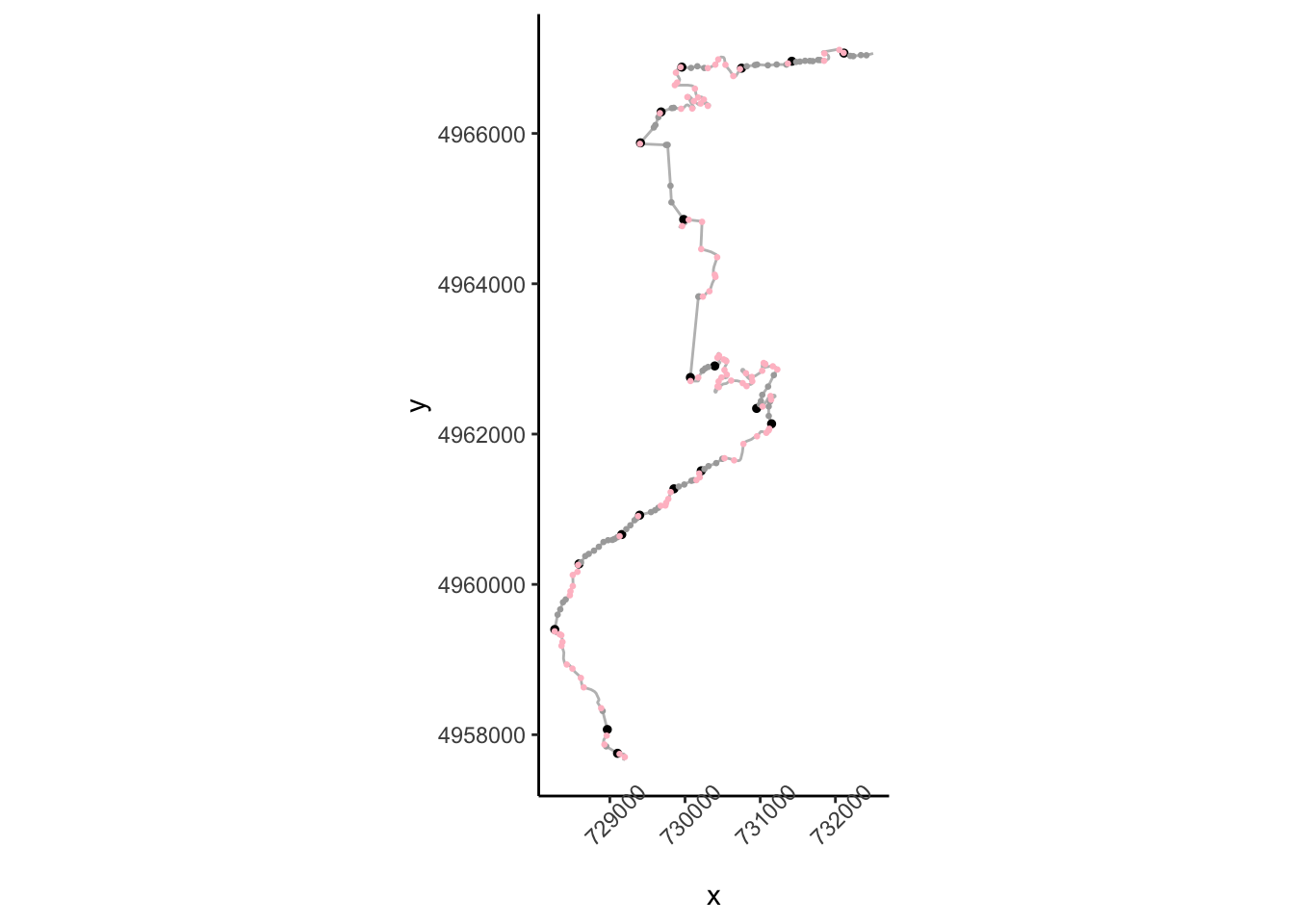## 20.6 Plotting Trajectory Segments

It will be convenient to be able ot plot segments of a trajectory for particular stretches of a route, so let’s create a function that helps us do that:

``````trj_segment_plot = function(trj, start, end,
x='x', y='y',
title='', fix_coords=TRUE,
rotate=NULL,

# Create the route distance filter limits
segment_filter = trj\$cum_dist >= start &
trj\$cum_dist <= end

# Filter the route
route_segment = trj[segment_filter,]

if (!is.null(rotate))
route_segment = TrajRotate(route_segment,
angle = rotate,
relative = TRUE)

# Generate the stylised route plot
g = ggplot(route_segment) +
geom_path(aes_string(x=x, y=y)) +
# Add a marker to show the start of the segment
aes_string(x=x, y=y)) +
theme_void()

title=as.character(title)
if (startsWith(title,'auto::')) {
title = stringr::str_split(title,'::')[]
title_ = paste0(start/1000, '-', end/1000, 'km')
if (title!='')
title = paste(title, title_)
else
title = title_
}

if (title!='')
g = g + ggtitle(title)

if (fix_coords)
g = g + coord_fixed()

g = g+ geom_point(aes_string(x=x, y=y),
color='red')
g
}``````

We can then plot a trajectory as follows, optionally rotatint the segment to a horizontal left-right orientation:

``````# Show a specified segment
trj_segment_plot(trj, 9000, 12500, title='auto::SS1', rotate=TRUE)``````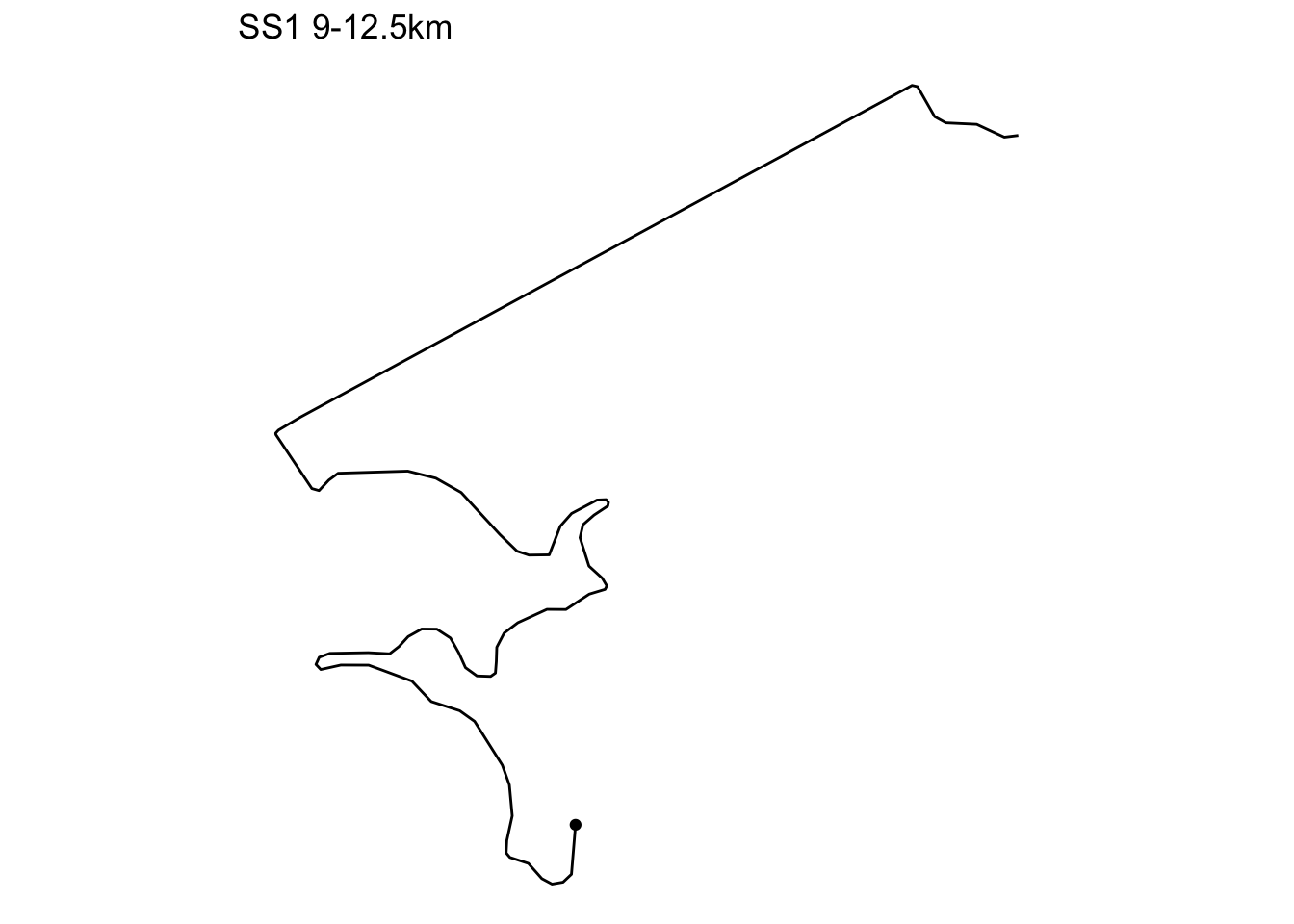### 20.6.1 Segmenting the Route

We can automate the production of a single chart that will display the route a section at a time. This requires segmenting the route, then for each segment, generating the plot, which we add to a list of plots.

``````# The final section goes to the end of the route
trj_segment_multiplot = function(trj, i, title='',
x='x', y='y',
final=FALSE,
segment_length = 1000,
prefix_dist = 20,
fix_coords=FALSE, rotate=NULL){

# Preface the start of the stage with a 20m lead
start_prefix = prefix_dist
start = segment_length*(i-1)-start_prefix
if (final)
end = Inf
else
end = (segment_length*i)

trj_segment_plot(trj, start, end,  x=x, y=y,
title=title,
fix_coords=fix_coords,
rotate=rotate,
}

# Create a list to hold each plot as a separate item
trj_segment_plots = list()

# How many kilometers does the route extend over?
length_1km = 1000
kms = floor(max(trj\$cum_dist)/length_1km)

# Iterate through each kilometer
for (i in 1:kms){
# Add each plot to the plot list
trj_segment_plots[[length(trj_segment_plots) + 1]] <-
trj_segment_multiplot(trj, i,
title=i, final=(i==kms),
fix_coords=TRUE, rotate=0,
}``````

We can use the `ggpubr::ggarrange()` function to plot the list of individual plots as a single image:

``````library(ggpubr)

gg = ggarrange(plotlist=trj_segment_plots,
ncol=5, nrow=ceiling(kms/4))

annotate_figure(gg,
top = text_grob(utm_routes[1,]\$Name, color = "black",
face = "bold", size = 14))``````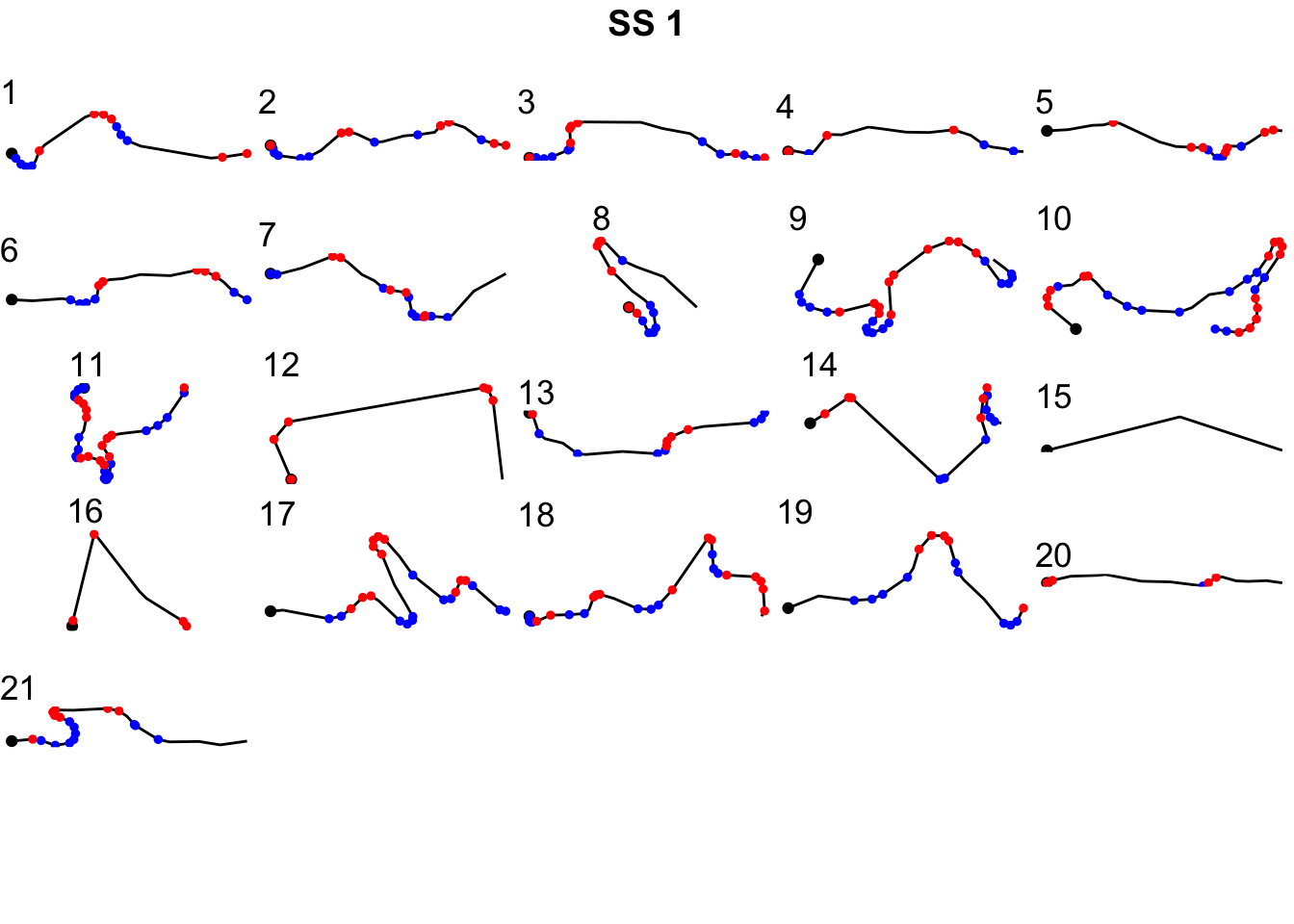### 20.6.2 Numbering Corners

To make it easier to refer to corners, can we use the firstish flag as a way of counting corners?

``````trj_corners = trj[trj\$firstish,]
trj_corners\$corner_num = cumsum(trj_corners\$firstish)

zoom1_corners = trj_corners[trj_corners\$y>4964000 & trj_corners\$y<4965000,]

ggplot(data=trj[zoom1, ],
aes(x=x, y=y)) + geom_path(color='grey') + coord_sf() +
geom_text(data= zoom1_corners,
aes(x=x, y=y, label=corner_num),
size=3) +
theme(axis.text.x = element_text(angle = 45))``````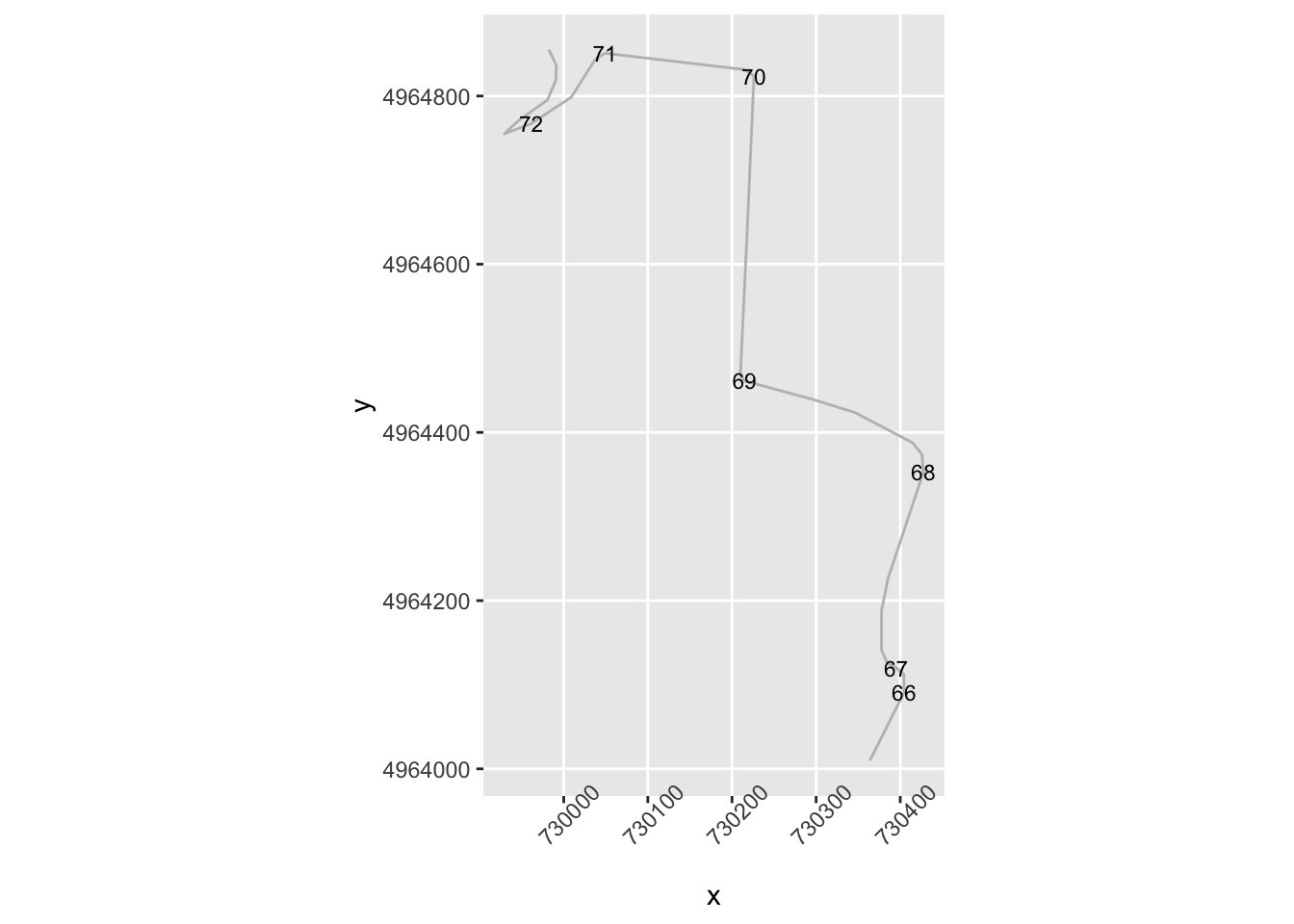## 20.7 Categorising Corners Using `rLFT` Convexity

The previous approach works to a certain extent with the limited dataset we tested it against but it feels rather contrived. Generally, just by looking at a curve we get a feel for how tight it is, rather than having to apply all sorts of decision-making rules and logical reasoning. Is there a simple metric we can use?

One approach might be to consider using convexity measure, such as the one provided by the `rLFT` package. Let’s see how that might work using the `rLFT::bct()` (bounded convexity tool) function. This function finds the curvature at regular points along a track, in the following example, every 10 meters:

``````library(rLFT)

stepdist = 10
window = 20
route_convexity <- bct(utm_routes[1,],
# distance between measurements
step = stepdist,
window = window, ridName = "Name")``````
``````##    user  system elapsed
##   0.172   0.034   0.208
##  "Features skipped due to size: "
## logical(0)``````
``head(route_convexity)``
``````##   FID  RID MidMeas WindowSize RawConvexity ConvexityIndex Sinuosity Midpoint_X
## 1   1 SS 1      10         20        0.000          0.000     0.500   729181.8
## 2   1 SS 1      20         20       -0.040         -0.004     0.500   729186.1
## 3   1 SS 1      30         20       -0.014         -0.001     0.500   729190.4
## 4   1 SS 1      40         20        0.000          0.000     0.500   729194.6
## 5   1 SS 1      50         20       -1.739         -0.174     0.509   729198.8
## 6   1 SS 1      60         20       -0.093         -0.009     0.501   729199.6
##   Midpoint_Y
## 1    4957667
## 2    4957676
## 3    4957685
## 4    4957694
## 5    4957703
## 6    4957713``````

Recalling the previous chapter:

``````corner_conv = 0.1

tight_corners = route_convexity[abs(route_convexity\$ConvexityIndex)>corner_conv,]
tight_corners_zoom1 = tight_corners\$Midpoint_Y>4964000 & tight_corners\$Midpoint_Y<4965000

ggplot(data=trj[zoom1, ],
aes(x=x, y=y)) + geom_path(color='grey') + coord_sf() +
geom_text(data=tight_corners[tight_corners_zoom1,],
aes(label = ConvexityIndex,
x=Midpoint_X, y=Midpoint_Y),
size=2) +
geom_point(data=tight_corners[tight_corners_zoom1,],
aes(x=Midpoint_X, y=Midpoint_Y,
color= (ConvexityIndex>0) ), size=1) +
theme_classic()+
theme(axis.text.x = element_text(angle = 45))``````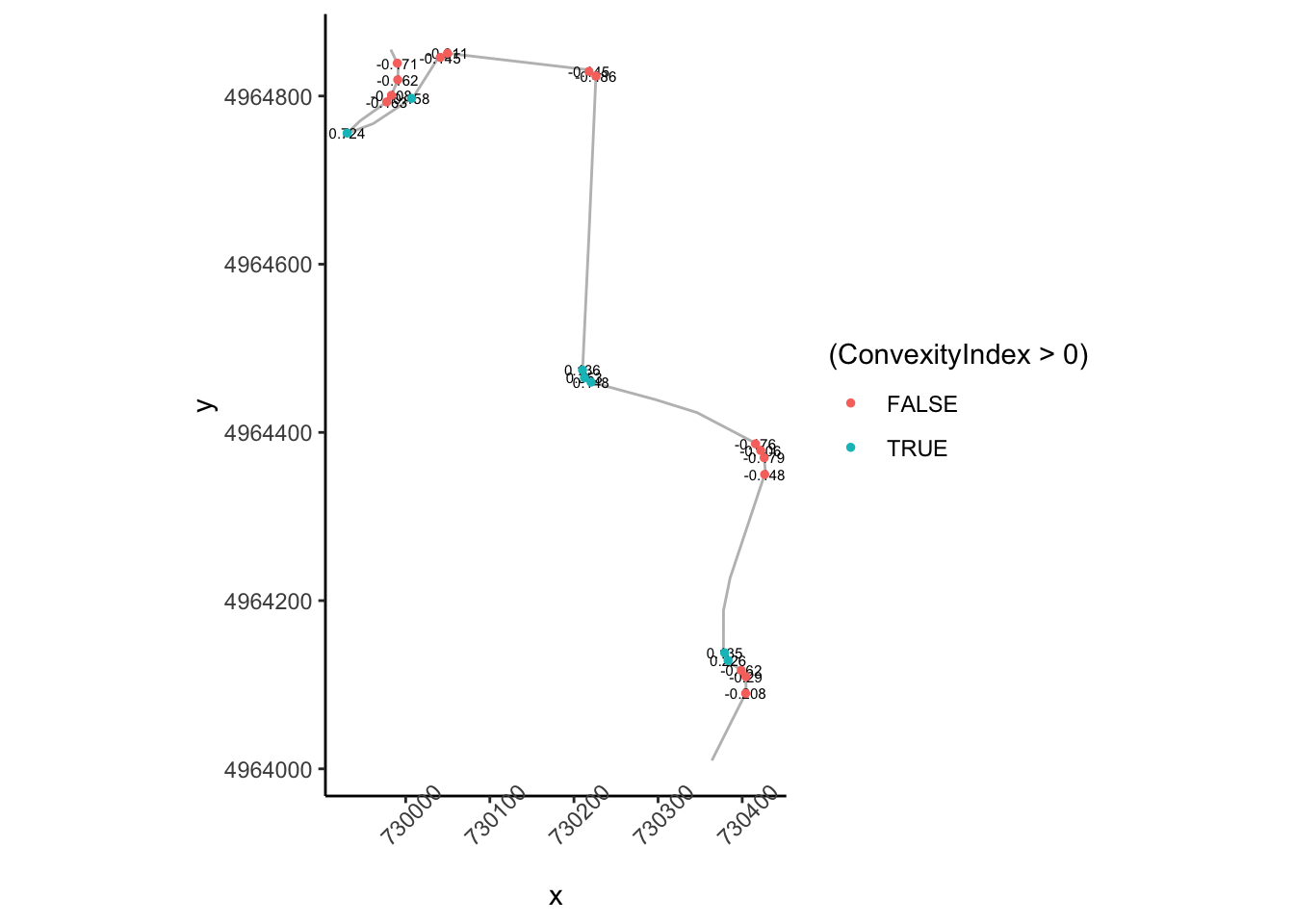So the absolute convexity index can help us find corners but we still need to do some work to find find the start of a corner.

How does that top right area look?

``````tight_corners_zoom2 = tight_corners_zoom1 & tight_corners\$Midpoint_X<730100

ggplot(data=trj[zoom2,],
aes(x=x, y=y)) + geom_path(color='grey') + coord_sf() +
ggrepel::geom_text_repel(data=tight_corners[tight_corners_zoom2,],
aes(label = ConvexityIndex,
x=Midpoint_X, y=Midpoint_Y),
size=3) +
geom_point(data=tight_corners[tight_corners_zoom2,],
aes(x=Midpoint_X, y=Midpoint_Y,
color= (ConvexityIndex>0) ), size=1) +
theme_classic()+
theme(axis.text.x = element_text(angle = 45))``````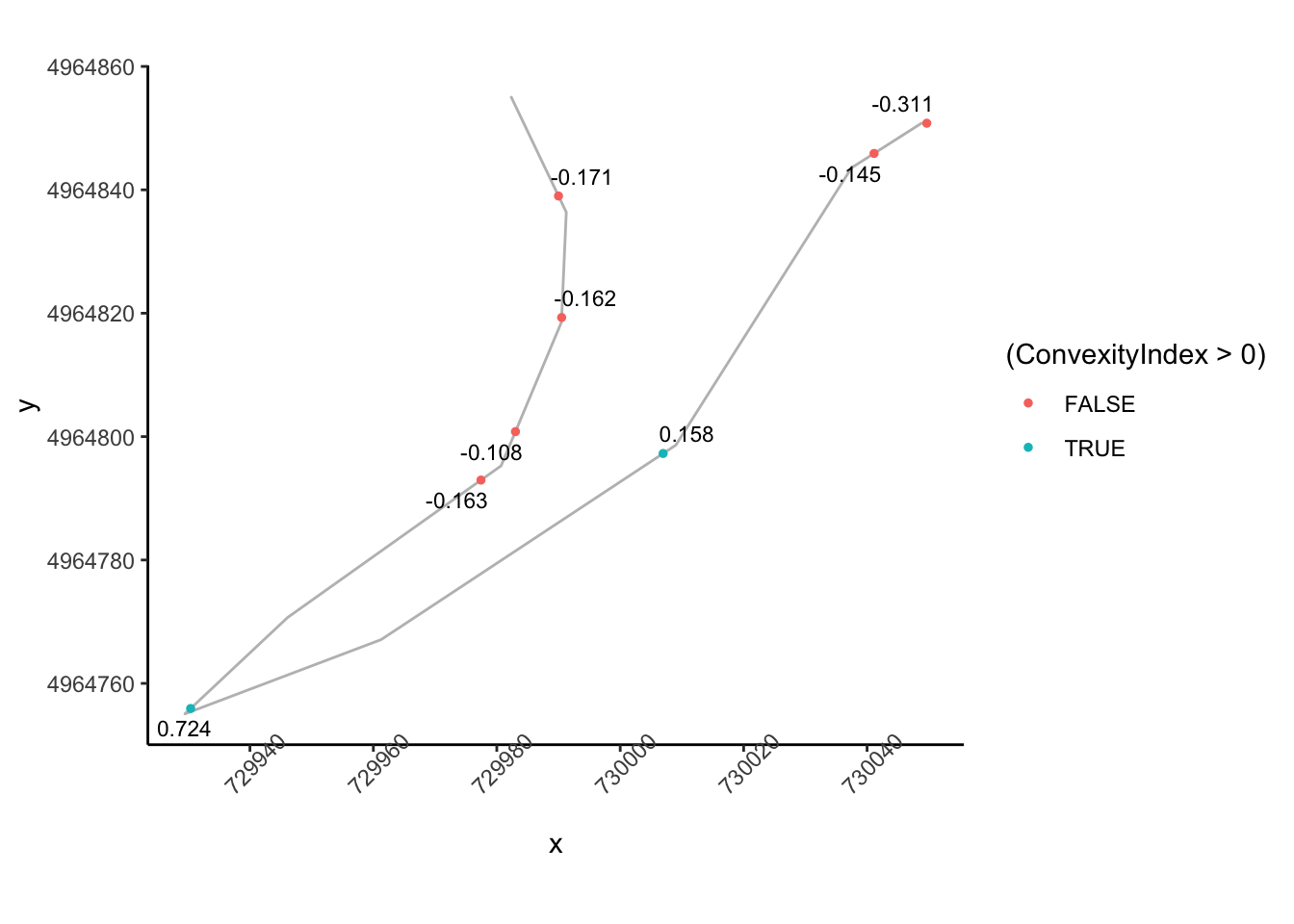So we can probably apply a similar logic to previously to group these and identify the corner entry node, although we need to tweak the rules slightly so that we are identifying a corner entry node that is far from a previous tight corner node:

``````cornerer = function (df, slight_conv=0.01, closeby=25){
df %>%
mutate(dirChange = sign(ConvexityIndex) != sign(lag(ConvexityIndex))) %>%
mutate(straightish =  (abs(ConvexityIndex) < slight_conv)) %>%
mutate(nearby =  dist < closeby) %>%
mutate(firstish = !straightish &
((nearby & !lag(straightish) & lag(dirChange)) |
# We don't want the previous node nearby
(!lag(nearby)) ) & !lag(nearby) )
}

tight_corners = cornerer(tight_corners)``````

Let’s see how it looks. We can also label nodes showing how far away the next node is:

``````ggplot(data=trj[zoom1,],
aes(x=x, y=y)) + geom_path(color='grey') + coord_sf() +
ggrepel::geom_text_repel(data=tight_corners[tight_corners_zoom1,],
aes(label = dist,
x=Midpoint_X, y=Midpoint_Y),
size=3) +
geom_point(data=tight_corners[tight_corners_zoom1,],
aes(x=Midpoint_X, y=Midpoint_Y,
color= (firstish) ), size=1) +
theme_classic()+
theme(axis.text.x = element_text(angle = 45))``````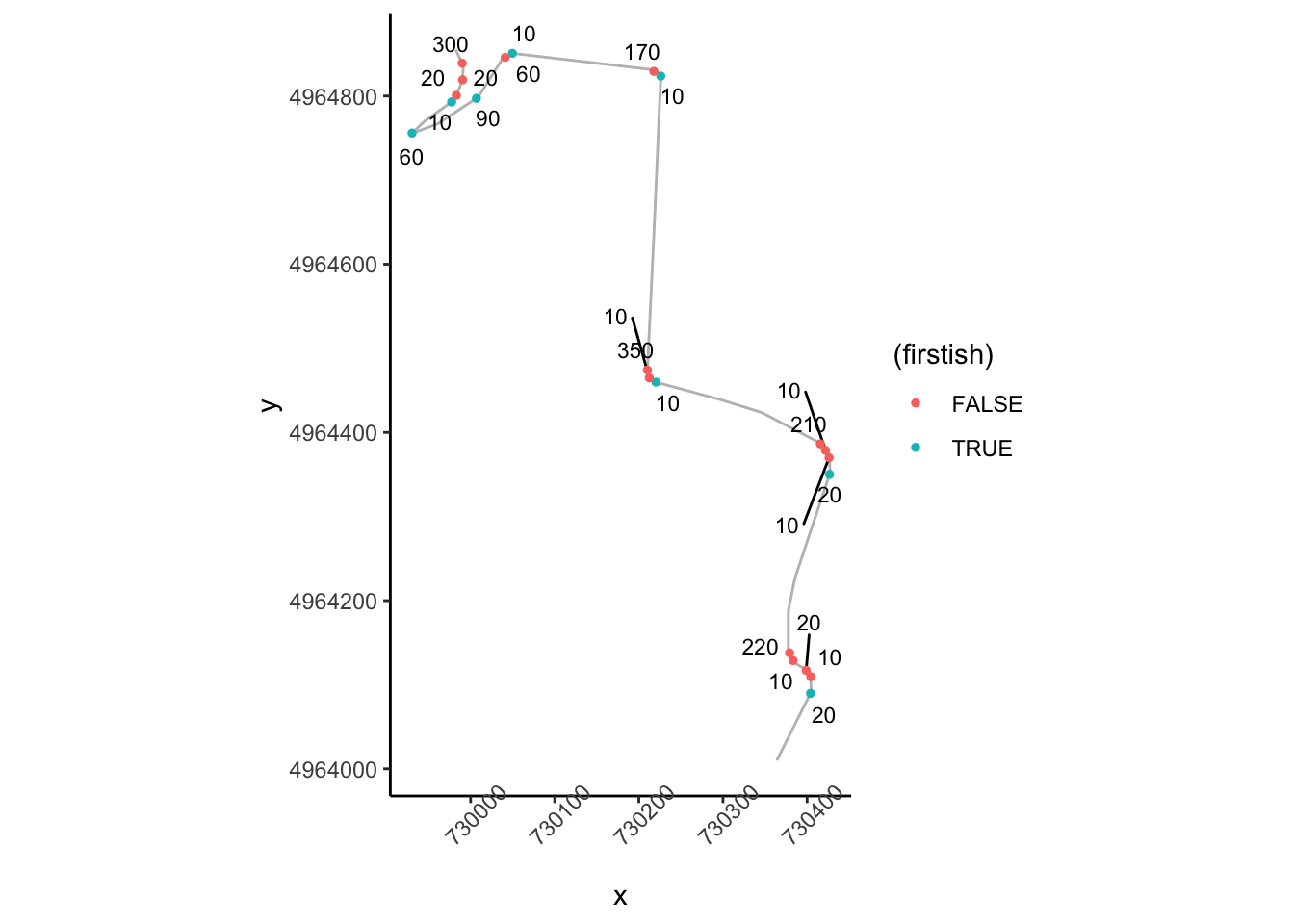## 20.8 Stage Segmenting

One of the handy things about the `rLFT::bct()` is that it can chunk the route into very short segments. This provides us with an easy way to index into the route to generate a view over a particular section.

For example, we can view the route over specific sections given a start and finish distance into the route. Note that the route will be discretised to step lengths used by the `rLFT::bct()` function and a stylised route will be approximated along step midmeasure points:

``````segment_plot = function(start, end, title='', fix_coords=TRUE) {

# Create the route distance filter limits
segment_filter = route_convexity\$MidMeas >= start &
route_convexity\$MidMeas <= end

# Filter the route
route_segment = route_convexity[segment_filter,]

# Generate the stylised route plot
g= ggplot(route_segment) +
geom_path(aes(x=Midpoint_X, y=Midpoint_Y)) +
aes(x=Midpoint_X, y=Midpoint_Y)) +
theme_void()

if (title!='')
g = g+ggtitle(title)

if (fix_coords)
g=g+coord_fixed()

g
}

# Show the first 1000m
segment_plot(0, 1000)``````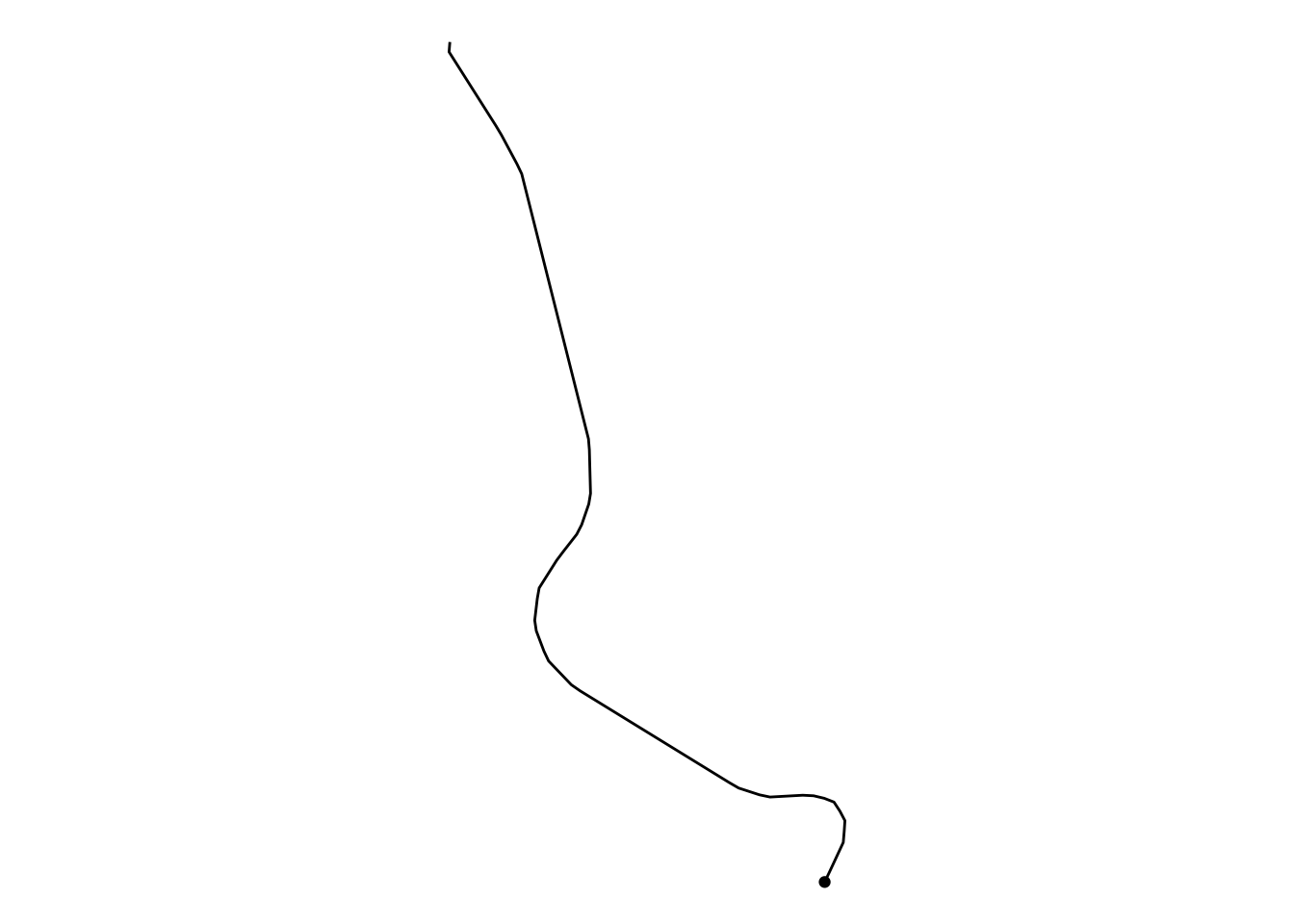We can automate the creation of route sections every kilometer and create a list of plots, one per kilometer section:

``````segment_length = 1000
step_length=10

kms = floor(max(route_convexity\$MidMeas)/segment_length)

# The final section goes to the end of the route
segment_multiplot = function(i, final=FALSE){
# Preface the start of the stage with a 20m lead
start_prefix = 2*step_length
start = segment_length*(i-1)-start_prefix
if (final)
end = Inf
else
end = (segment_length*i)

segment_plot(start, end, i, fix_coords=TRUE)
}

# Create a list to hold each plot as a separate item
segment_plots = list()

# Iterate through each kilometer
for (i in 1:kms){
# Add each plot to the plot list
segment_plots[[length(segment_plots) + 1]] <-
segment_multiplot(i, final=(i==kms))
}``````

We can now use the `ggpubr::ggarrange()` function to display all the stage route sections in a single plot:

``````ggarrange(plotlist=segment_plots,
ncol=5, nrow=ceiling(kms/4))``````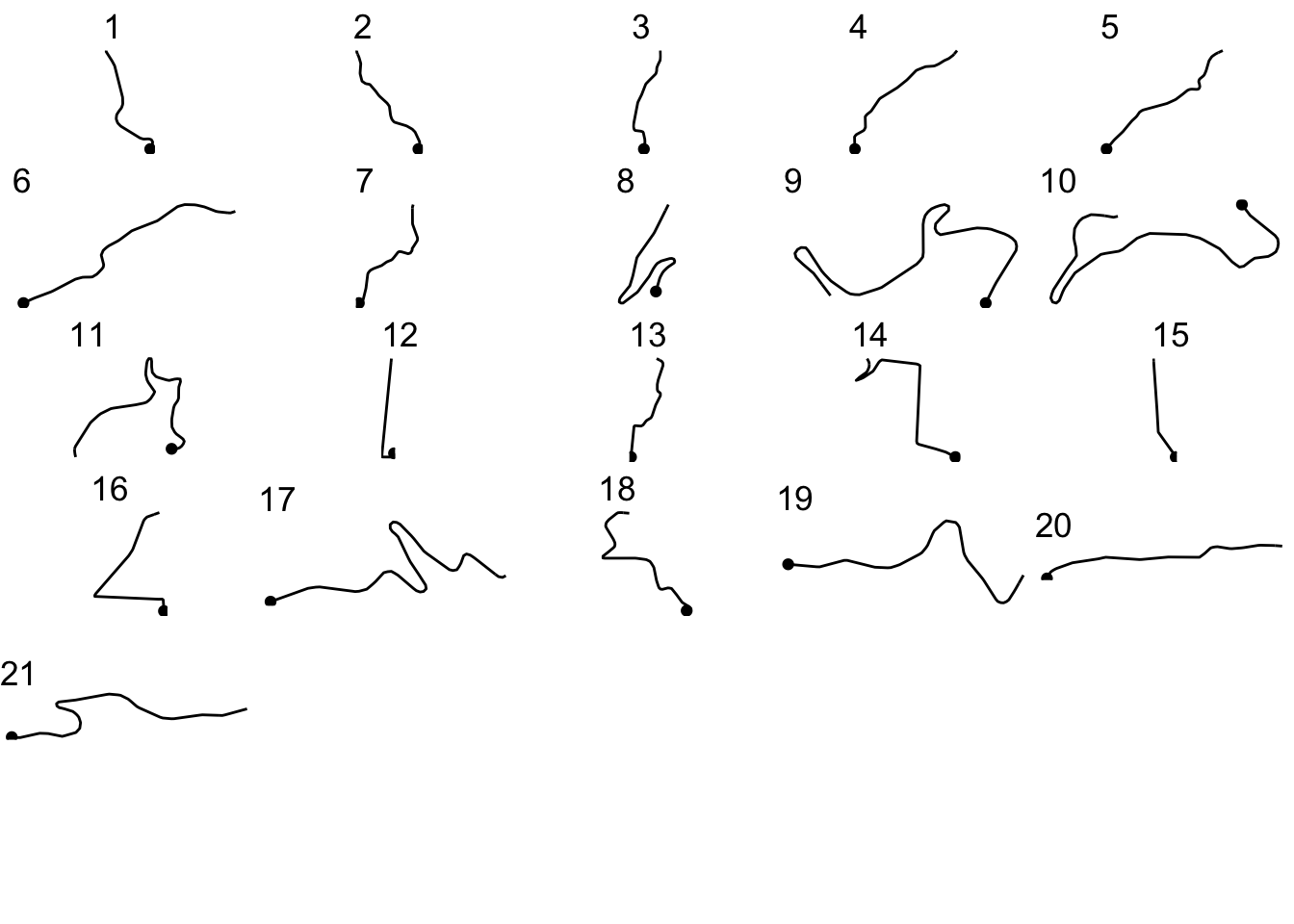We can use a similar approach to create a more general function that will allow us to plot either the route, or generate a plot for each segment showing the convexity against distance into the segment:

``````# Simple function to get a route segment
get_route_segment = function (route, start, end){
segment_filter = route\$MidMeas >= start &
route\$MidMeas <= end

route[segment_filter,]
}

segment_plot2 = function(route, start, end, title='',
typ='route', themevoid=TRUE){

# Get route segment
route_segment = get_route_segment(route, start, end)

# Create plot base
g = ggplot(route_segment)

if (typ=='convexity') {
g = g + geom_bar(aes(x=MidMeas,
y=-ConvexityIndex,
col=(ConvexityIndex>0)),
stat='identity', show.legend = FALSE)

} else {
# plot route
g = g + geom_path(aes(x=Midpoint_X, y=Midpoint_Y)) +
aes(x=Midpoint_X, y=Midpoint_Y))
}

if (title!='')
g = g + ggtitle(title)

if (themevoid)
g = g + theme_void()

g
}``````

Let’s see what a convexity report for the first kilometer looks like:

``segment_plot2(route_convexity, 0, 1000, typ='convexity')``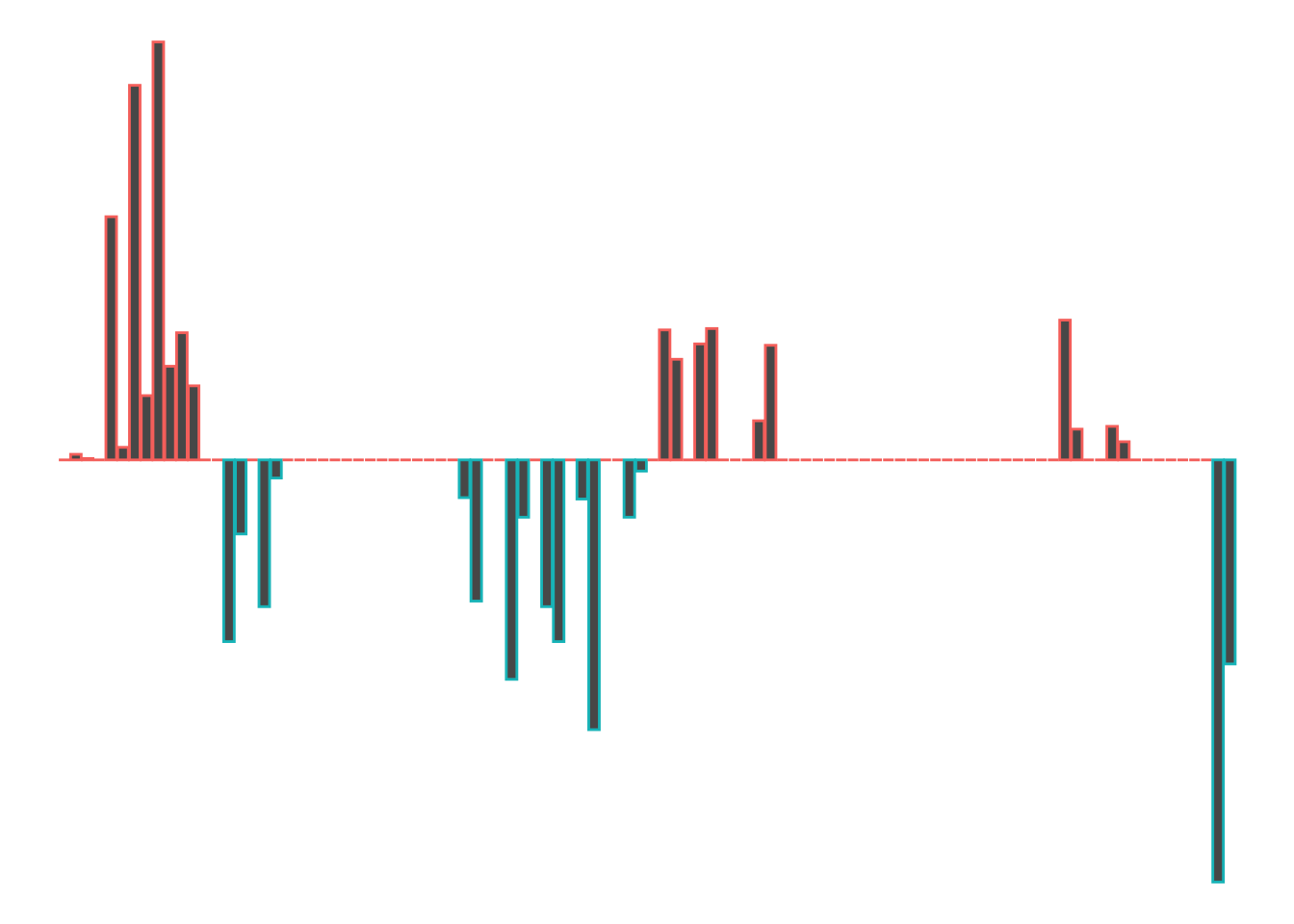So we start off with a left (port-red-left!), a slight right, a straight, a right that tightens, a left, a straight, a nudge left and then end the kilometer with a tight right.

Let’s remind ourselves to see if that looks about right:

``segment_plot2(route_convexity, 0, 1000, typ='route')``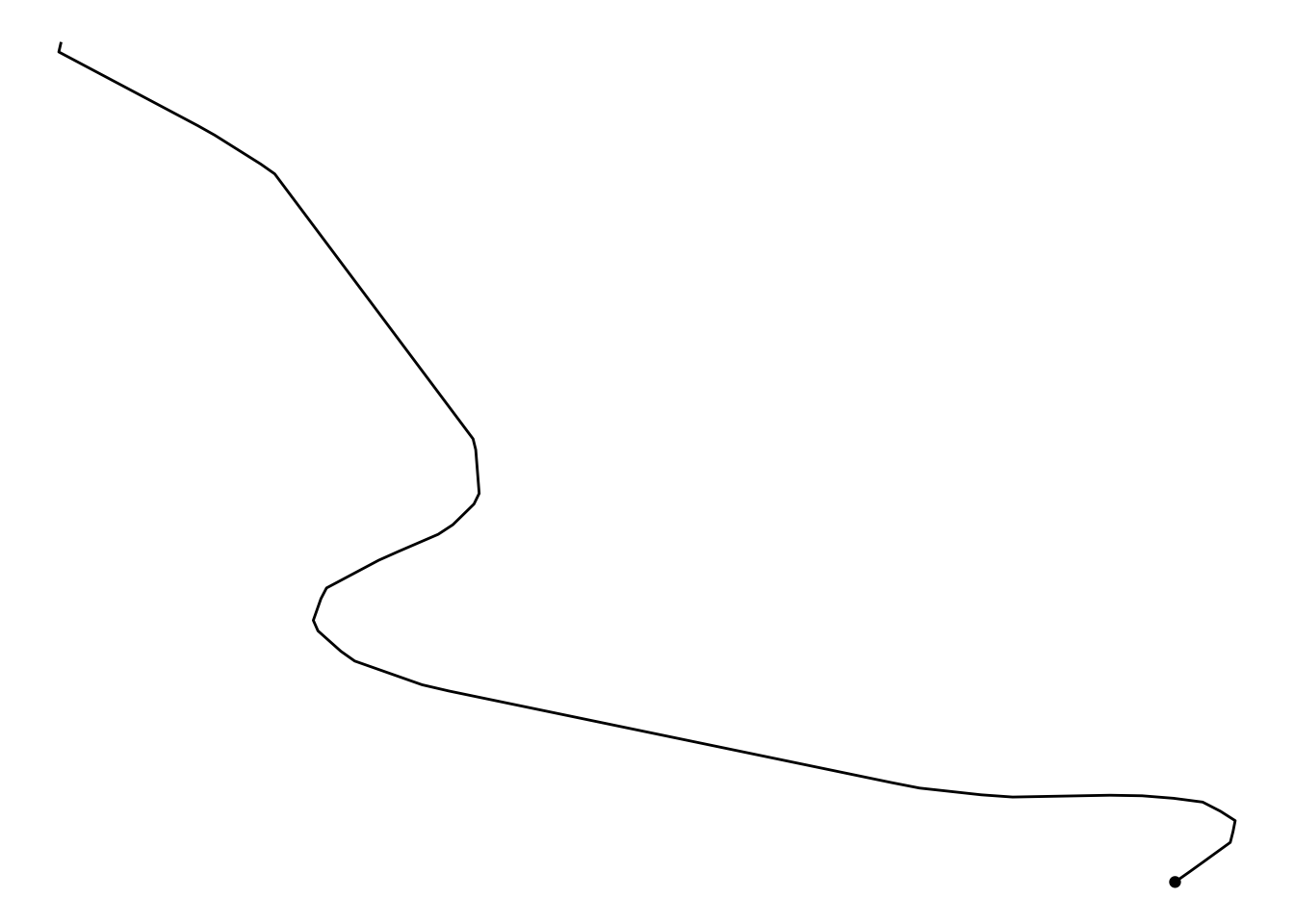We can use a similar approach before to automate the collection of multiple plots for each stretch of the stage:

``````segment_multiplot2 = function(route, i, final=FALSE, typ='route'){
start = segment_length*(i-1)-step_length
if (final)
end = Inf
else
end = (segment_length*i)

segment_plot2(route, start, end, i, typ )
}``````

We can now generate a plot of either the route sections, or the convexity based on distance into the route section:

``````segment_plots2 = list()

# Iterate through each kilometer
for (i in 1:(kms-1)){
segment_plots2[[length(segment_plots2) + 1]] <-
segment_multiplot2(route_convexity, i,  final= (i==kms),
typ = 'convexity')
}

ggpubr::ggarrange(plotlist=segment_plots2,
ncol=5, nrow=ceiling(kms/4))``````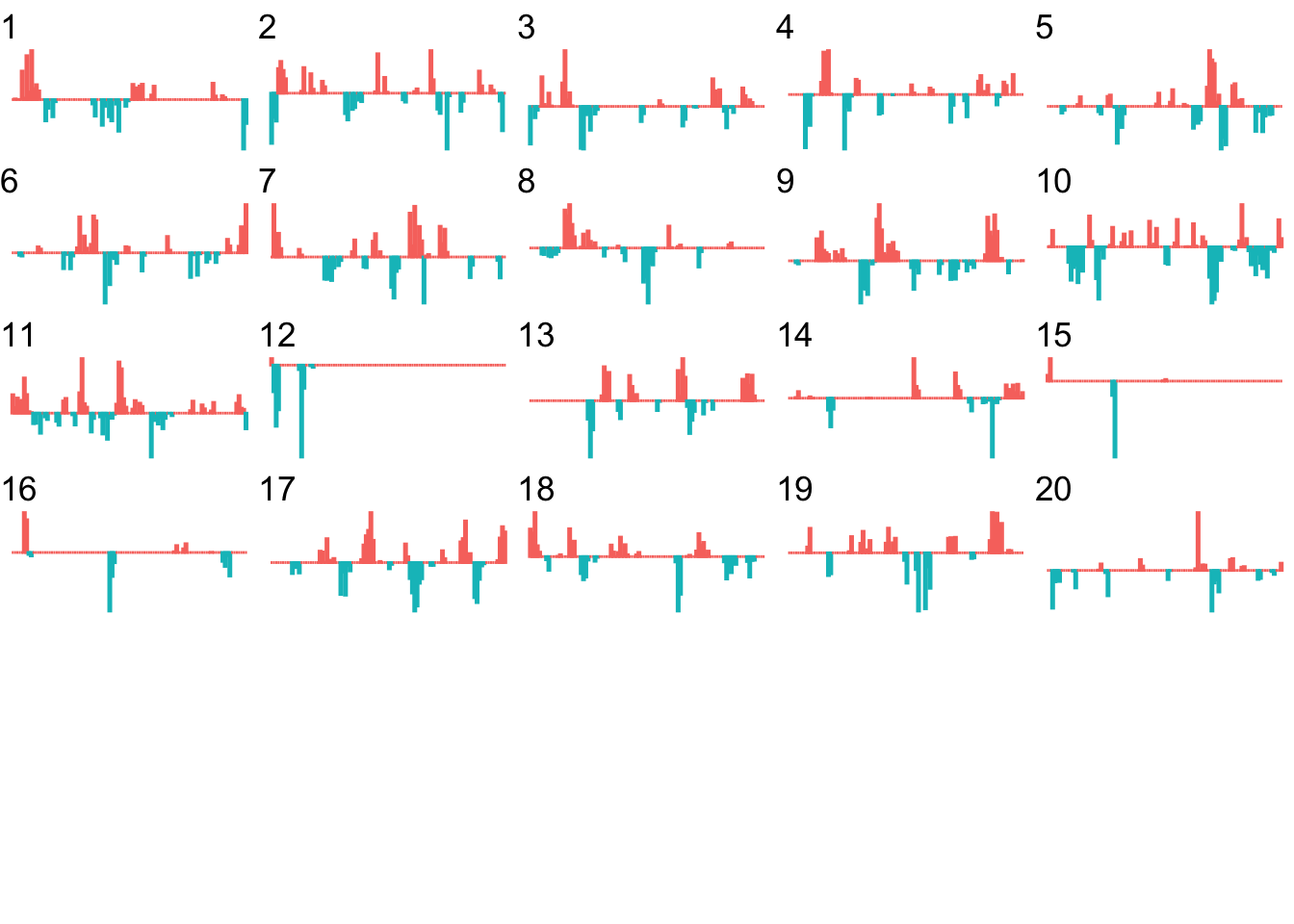At a glance, we can now start to identify segments of the route that contain particular sorts of feature.

For example, we can readily see that kilometers 12, 15 and 16 include significant straight stretches.

## 20.9 Corner Severity Numbering

Although they may differ in the specifics, all rally pace note systems find some way to categorise the corner severity (also referred to as the turn intensity). Many systems use a convention that numbers corner severity with a value from 1 to 6, where 1 is the most severe and 6 is the least severe; alternatively, therallydriver.com pacenotes system, (which has the added benefit of being well documented in the To See the Invisible tutorial series published by the system’s creator, David Nafría), uses a range from 1 to 10.

Variations on the following diagram are often used to illustrate how the numbers scheme applies to different severity corners.

``````library(ggforce)
# ggforce::geom_arc

rallynotes = data.frame(n=1:10,
r=c(10, 15, 20, 27.5, 35,
45, 60, 77.5, 100, 175))

rallynotes_arcs <- data.frame(
start =  -pi/2,
end = mapply(min, 50/rallynotes\$r, pi/2),
r = rallynotes\$r,
n = rallynotes\$n
)

# Plot a representation of corner severity along the route
ggplot(rallynotes_arcs) +
geom_arc_bar(aes(x0 = -15+r-6*n, y0 = 0, r0 = r,
r = r+2, start = start,
end = end,fill = factor(n),
color = factor(n)), show.legend=FALSE) +
geom_text(aes(label=n, x=-15-6*n , y=-10),
size=3, hjust='right') +
coord_fixed()+
theme_void() ``````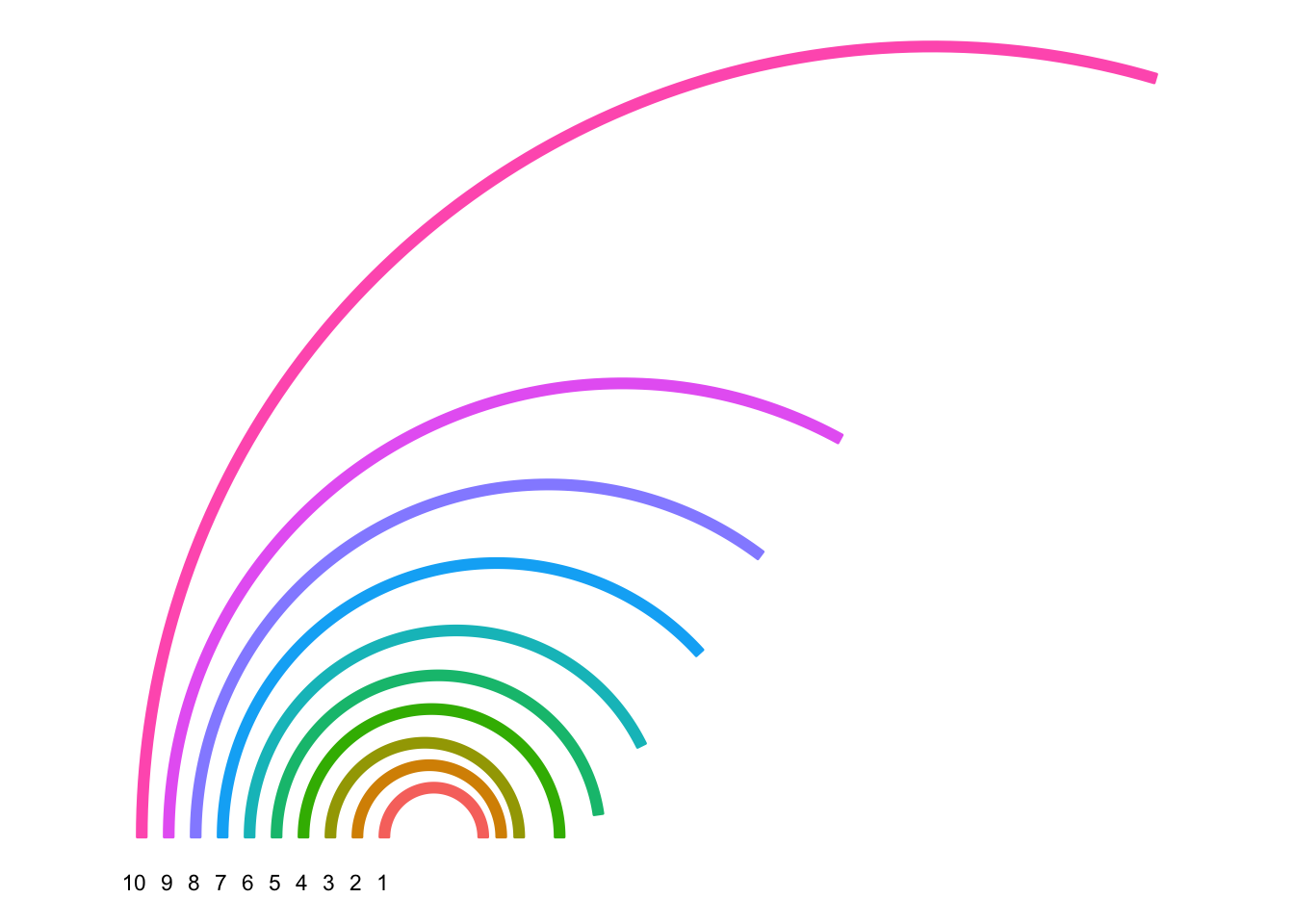Note that with increasing number, the radius of curvature (that is, the radius of the circle describing the corner) also increases. This gives us one way of mapping from a measurement we can apply to a corner (such as its radius of curvature) to the turn severity number.

Two other properties of a corner that we might attempt to describe are the length of the corner either as a linear distance (20m, for example) or as an angular distance (45 degrees, for example). The “distance” might also map on to a descriptive term, such as “long”, as in “long right”. Descriptive terms are more ambiguous because they are normative: they assume a “standard” length corner in comparison with which a “long” corner is longer.

``````curvature = function(x,y){
#729181.8, 729186.1, 729190.4
#4957667 , 4957676, 4957685
tryCatch({
# circlefit gives an error if we pass a straight line
# Also hide the print statement in circlefit
pracma::circlefit(x,y)
},
error = function(err) {
# For a straight, return the first co-ord and Inf diameter
# Alternatively, pass zero diameter?
c(x, y, Inf)})
}

curvature2 = function(x1, x2, x3, y1, y2, y3){
curvature(c(x1, x2, x3), c(y1, y2, y3))
}

curvatures = Vectorize(curvature2)

curvatures2 = function(route,
x='Midpoint_X', y='Midpoint_Y') {

curvatures(lag(route[[x]]),
route[[x]],
lag(route[[y]]),
route[[y]],
}``````
``route_convexity\$turn_curvature = curvatures2(route_convexity)``

Preview the result:

``head(route_convexity, c('ConvexityIndex', 'turn_curvature' ))``
``````##     FID  RID MidMeas WindowSize RawConvexity ConvexityIndex Sinuosity Midpoint_X
## 1     1 SS 1      10         20        0.000          0.000     0.500   729181.8
## 2     1 SS 1      20         20       -0.040         -0.004     0.500   729186.1
## 3     1 SS 1      30         20       -0.014         -0.001     0.500   729190.4
## 4     1 SS 1      40         20        0.000          0.000     0.500   729194.6
## 5     1 SS 1      50         20       -1.739         -0.174     0.509   729198.8
## 6     1 SS 1      60         20       -0.093         -0.009     0.501   729199.6
## 7     1 SS 1      70         20       -2.684         -0.268     0.527   729200.3
## 8     1 SS 1      80         20       -0.464         -0.046     0.507   729195.8
## 9     1 SS 1      90         20       -2.989         -0.299     0.537   729190.3
## 10    1 SS 1     100         20       -0.672         -0.067     0.512   729181.4
## 11    1 SS 1     110         20       -0.907         -0.091     0.505   729171.7
## 12    1 SS 1     120         20       -0.527         -0.053     0.503   729161.8
## 13    1 SS 1     130         20        0.000          0.000     0.500   729151.8
## 14    1 SS 1     140         20        0.000          0.000     0.500   729141.8
## 15    1 SS 1     150         20        1.303          0.130     0.508   729131.8
## 16    1 SS 1     160         20        0.531          0.053     0.504   729122.2
## 17    1 SS 1     170         20        0.000          0.000     0.500   729112.7
## 18    1 SS 1     180         20        1.053          0.105     0.503   729103.1
## 19    1 SS 1     190         20        0.127          0.013     0.501   729094.5
## 20    1 SS 1     200         20        0.000          0.000     0.500   729086.0
## 21    1 SS 1     210         20        0.000          0.000     0.500   729077.5
## 22    1 SS 1     220         20        0.000          0.000     0.500   729069.0
## 23    1 SS 1     230         20        0.000          0.000     0.500   729060.5
## 24    1 SS 1     240         20        0.000          0.000     0.500   729051.9
## 25    1 SS 1     250         20        0.000          0.000     0.500   729043.4
## 26    1 SS 1     260         20        0.000          0.000     0.500   729034.9
## 27    1 SS 1     270         20        0.000          0.000     0.500   729026.4
## 28    1 SS 1     280         20        0.000          0.000     0.500   729017.9
## 29    1 SS 1     290         20        0.000          0.000     0.500   729009.3
## 30    1 SS 1     300         20        0.000          0.000     0.500   729000.8
## 31    1 SS 1     310         20        0.000          0.000     0.500   728992.3
## 32    1 SS 1     320         20        0.000          0.000     0.500   728983.8
## 33    1 SS 1     330         20        0.000          0.000     0.500   728975.3
## 34    1 SS 1     340         20        0.000          0.000     0.500   728966.8
## 35    1 SS 1     350         20        0.270          0.027     0.502   728958.2
## 36    1 SS 1     360         20        1.009          0.101     0.504   728950.1
## 37    1 SS 1     370         20        0.000          0.000     0.500   728943.2
## 38    1 SS 1     380         20        0.000          0.000     0.500   728936.3
## 39    1 SS 1     390         20        1.574          0.157     0.510   728929.3
## 40    1 SS 1     400         20        0.412          0.041     0.504   728925.1
## 41    1 SS 1     410         20        0.000          0.000     0.500   728921.6
## 42    1 SS 1     420         20        1.050          0.105     0.510   728918.0
## 43    1 SS 1     430         20        1.304          0.130     0.512   728916.6
## 44    1 SS 1     440         20        0.000          0.000     0.500   728917.8
## 45    1 SS 1     450         20        0.280          0.028     0.503   728919.0
## 46    1 SS 1     460         20        1.927          0.193     0.512   728920.7
## 47    1 SS 1     470         20        0.000          0.000     0.500   728926.0
## 48    1 SS 1     480         20        0.000          0.000     0.500   728931.4
## 49    1 SS 1     490         20        0.409          0.041     0.501   728936.7
## 50    1 SS 1     500         20        0.084          0.008     0.500   728942.7
## 51    1 SS 1     510         20        0.000          0.000     0.500   728948.9
## 52    1 SS 1     520         20       -0.930         -0.093     0.506   728955.0
## 53    1 SS 1     530         20       -0.716         -0.072     0.505   728959.5
## 54    1 SS 1     540         20        0.000          0.000     0.500   728962.8
## 55    1 SS 1     550         20       -0.830         -0.083     0.506   728966.0
## 56    1 SS 1     560         20       -0.940         -0.094     0.506   728967.6
## 57    1 SS 1     570         20        0.000          0.000     0.500   728967.4
## 58    1 SS 1     580         20        0.000          0.000     0.500   728967.1
## 59    1 SS 1     590         20        0.000          0.000     0.500   728966.9
## 60    1 SS 1     600         20       -0.280         -0.028     0.501   728966.6
## 61    1 SS 1     610         20       -0.818         -0.082     0.503   728965.8
## 62    1 SS 1     620         20        0.000          0.000     0.500   728963.3
## 63    1 SS 1     630         20        0.000          0.000     0.500   728960.9
## 64    1 SS 1     640         20        0.000          0.000     0.500   728958.4
## 65    1 SS 1     650         20        0.000          0.000     0.500   728956.0
## 66    1 SS 1     660         20        0.000          0.000     0.500   728953.6
## 67    1 SS 1     670         20        0.000          0.000     0.500   728951.1
## 68    1 SS 1     680         20        0.000          0.000     0.500   728948.7
## 69    1 SS 1     690         20        0.000          0.000     0.500   728946.2
## 70    1 SS 1     700         20        0.000          0.000     0.500   728943.8
## 71    1 SS 1     710         20        0.000          0.000     0.500   728941.4
## 72    1 SS 1     720         20        0.000          0.000     0.500   728938.9
## 73    1 SS 1     730         20        0.000          0.000     0.500   728936.5
## 74    1 SS 1     740         20        0.000          0.000     0.500   728934.0
## 75    1 SS 1     750         20        0.000          0.000     0.500   728931.6
## 76    1 SS 1     760         20        0.000          0.000     0.500   728929.1
## 77    1 SS 1     770         20        0.000          0.000     0.500   728926.7
## 78    1 SS 1     780         20        0.000          0.000     0.500   728924.3
## 79    1 SS 1     790         20        0.000          0.000     0.500   728921.8
## 80    1 SS 1     800         20        0.000          0.000     0.500   728919.4
## 81    1 SS 1     810         20        0.000          0.000     0.500   728916.9
## 82    1 SS 1     820         20        0.000          0.000     0.500   728914.5
## 83    1 SS 1     830         20        0.000          0.000     0.500   728912.1
## 84    1 SS 1     840         20        0.000          0.000     0.500   728909.6
## 85    1 SS 1     850         20        0.000          0.000     0.500   728907.2
## 86    1 SS 1     860         20       -0.995         -0.100     0.504   728904.7
## 87    1 SS 1     870         20       -0.225         -0.022     0.501   728900.4
## 88    1 SS 1     880         20        0.000          0.000     0.500   728895.7
## 89    1 SS 1     890         20        0.000          0.000     0.500   728891.0
## 90    1 SS 1     900         20       -0.236         -0.024     0.500   728886.3
## 91    1 SS 1     910         20       -0.132         -0.013     0.500   728881.1
## 92    1 SS 1     920         20        0.000          0.000     0.500   728875.8
## 93    1 SS 1     930         20        0.000          0.000     0.500   728870.4
## 94    1 SS 1     940         20        0.000          0.000     0.500   728865.1
## 95    1 SS 1     950         20        0.000          0.000     0.500   728859.7
## 96    1 SS 1     960         20        0.000          0.000     0.500   728854.4
## 97    1 SS 1     970         20        0.000          0.000     0.500   728849.0
## 98    1 SS 1     980         20        0.000          0.000     0.500   728843.6
## 99    1 SS 1     990         20        3.018          0.302     0.551   728838.3
## 100   1 SS 1    1000         20        1.456          0.146     0.531   728839.0
##     Midpoint_Y turn_curvature
## 1      4957667            Inf
## 2      4957676            Inf
## 3      4957685            Inf
## 4      4957694            Inf
## 5      4957703       28.64928
## 6      4957713      534.95134
## 7      4957723       18.13793
## 8      4957731            Inf
## 9      4957740       16.00415
## 10     4957743            Inf
## 11     4957745            Inf
## 12     4957746            Inf
## 13     4957745            Inf
## 14     4957745            Inf
## 15     4957744       37.82928
## 16     4957746            Inf
## 17     4957749            Inf
## 18     4957752       47.36890
## 19     4957757            Inf
## 20     4957763            Inf
## 21     4957768            Inf
## 22     4957773            Inf
## 23     4957778            Inf
## 24     4957784            Inf
## 25     4957789            Inf
## 26     4957794            Inf
## 27     4957799            Inf
## 28     4957805            Inf
## 29     4957810            Inf
## 30     4957815            Inf
## 31     4957820            Inf
## 32     4957826            Inf
## 33     4957831            Inf
## 34     4957836            Inf
## 35     4957841            Inf
## 36     4957847       49.27605
## 37     4957854            Inf
## 38     4957861            Inf
## 39     4957869       31.34610
## 40     4957878            Inf
## 41     4957887            Inf
## 42     4957896       46.25097
## 43     4957906       37.24827
## 44     4957916            Inf
## 45     4957926      176.80500
## 46     4957936       25.66148
## 47     4957944            Inf
## 48     4957952            Inf
## 49     4957961            Inf
## 50     4957969            Inf
## 51     4957977            Inf
## 52     4957985       53.02203
## 53     4957993       68.89935
## 54     4958003            Inf
## 55     4958012       59.29952
## 56     4958022       52.34419
## 57     4958032            Inf
## 58     4958042            Inf
## 59     4958052            Inf
## 60     4958062      177.62492
## 61     4958072       60.87898
## 62     4958082            Inf
## 63     4958091            Inf
## 64     4958101            Inf
## 65     4958111            Inf
## 66     4958120            Inf
## 67     4958130            Inf
## 68     4958140            Inf
## 69     4958149            Inf
## 70     4958159            Inf
## 71     4958169            Inf
## 72     4958179            Inf
## 73     4958188            Inf
## 74     4958198            Inf
## 75     4958208            Inf
## 76     4958217            Inf
## 77     4958227            Inf
## 78     4958237            Inf
## 79     4958246            Inf
## 80     4958256            Inf
## 81     4958266            Inf
## 82     4958276            Inf
## 83     4958285            Inf
## 84     4958295            Inf
## 85     4958305            Inf
## 86     4958314       50.01758
## 87     4958323            Inf
## 88     4958332            Inf
## 89     4958341            Inf
## 90     4958350            Inf
## 91     4958358            Inf
## 92     4958367            Inf
## 93     4958375            Inf
## 94     4958384            Inf
## 95     4958392            Inf
## 96     4958401            Inf
## 97     4958409            Inf
## 98     4958417            Inf
## 99     4958426       15.11854
## 100    4958435       31.14539
##  [ reached 'max' / getOption("max.print") -- omitted 2007 rows ]``````
``````ggplot(route_convexity[1:10,], aes(x=Midpoint_X, y=Midpoint_Y)) +
geom_path() + geom_point(aes(color=turn_curvature))``````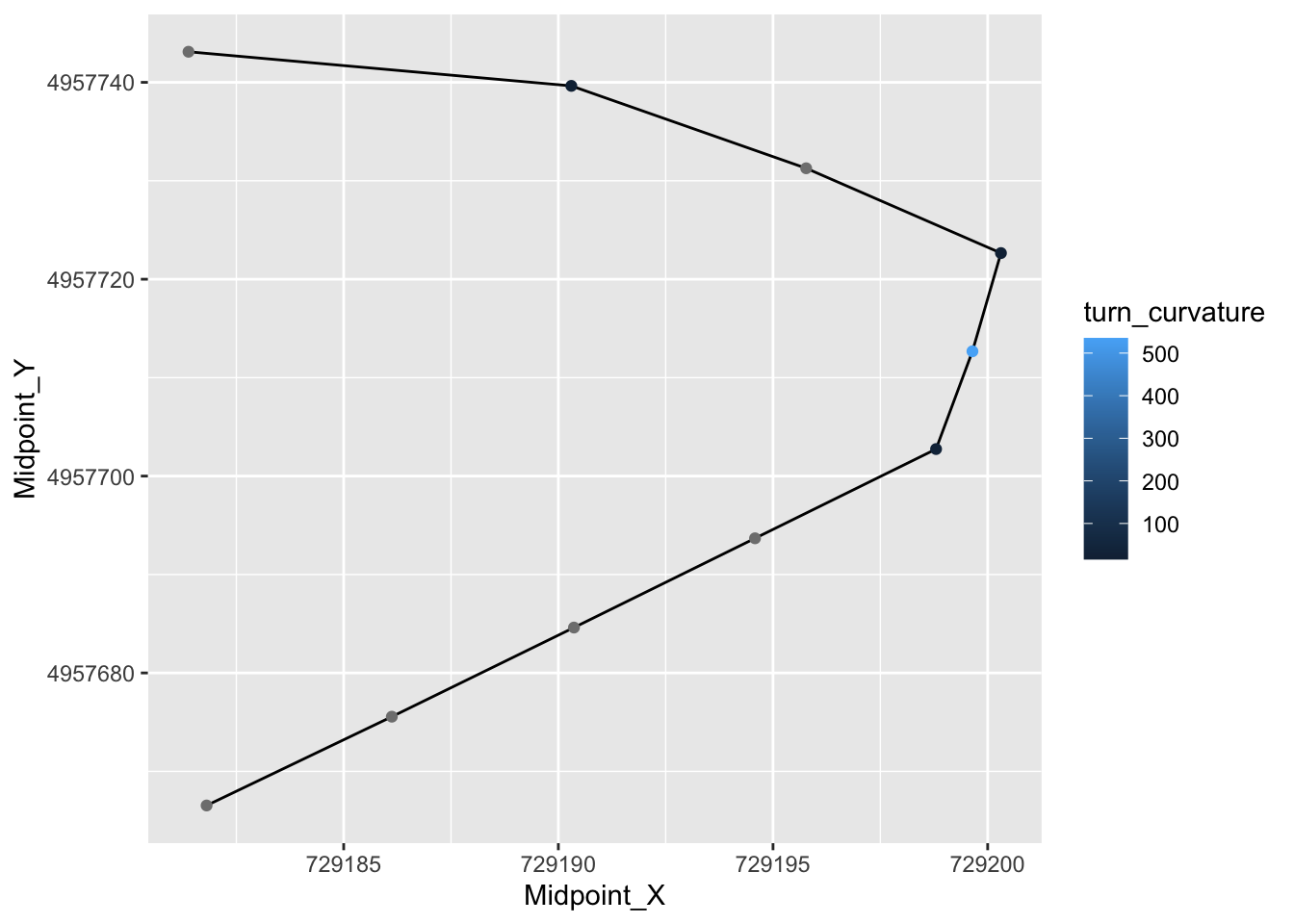``route_convexity[1:10,]``
``````##    FID  RID MidMeas WindowSize RawConvexity ConvexityIndex Sinuosity Midpoint_X
## 1    1 SS 1      10         20        0.000          0.000     0.500   729181.8
## 2    1 SS 1      20         20       -0.040         -0.004     0.500   729186.1
## 3    1 SS 1      30         20       -0.014         -0.001     0.500   729190.4
## 4    1 SS 1      40         20        0.000          0.000     0.500   729194.6
## 5    1 SS 1      50         20       -1.739         -0.174     0.509   729198.8
## 6    1 SS 1      60         20       -0.093         -0.009     0.501   729199.6
## 7    1 SS 1      70         20       -2.684         -0.268     0.527   729200.3
## 8    1 SS 1      80         20       -0.464         -0.046     0.507   729195.8
## 9    1 SS 1      90         20       -2.989         -0.299     0.537   729190.3
## 10   1 SS 1     100         20       -0.672         -0.067     0.512   729181.4
##    Midpoint_Y turn_curvature
## 1     4957667            Inf
## 2     4957676            Inf
## 3     4957685            Inf
## 4     4957694            Inf
## 5     4957703       28.64928
## 6     4957713      534.95134
## 7     4957723       18.13793
## 8     4957731            Inf
## 9     4957740       16.00415
## 10    4957743            Inf``````